Sébastien Labbé Sébastien Labbé 2019-06-09T19:56:34Z Blogofile http://www.slabbe.org/blogue/feed/atom/ Sébastien Labbé http://www.slabbe.org/blogue <![CDATA[Comment installer et utiliser RISE, une extension du notebook Jupyter pour faire des présentations]]> http://www.slabbe.org/blogue/2019/01/comment-installer-et-utiliser-rise-une-extension-du-notebook-jupyter-pour-faire-des-presentations 2019-01-24T10:37:00Z 2019-01-24T10:37:00Z

La semaine dernière, Jeroen Demeyer a fait une présentation lors de l'Atelier PARI/GP 2019 au sujet de cypari2.

La présentation de Jeroen consistait en des diapositives HTML où les calculs sont faits en direct (avec Jupyter) et où on peut les modifier en direct dans les diapositives. Impressionant! Tout cela grâce au package Python RISE.

Pour installer et utiliser RISE, une extension du Jupyter Notebook pour faire des présentations éditables, il ne suffit pas de l'installer il faut aussi recopier les css au bon endroit. Pour l'installer dans Sage, il suffit de faire:

sage -pip install rise
sage -sh
jupyter-nbextension install rise --py --sys-prefix

Après on peut consulter ce démo sur youtube et la documentation de RISE est ici.

]]>
Sébastien Labbé http://www.slabbe.org/blogue <![CDATA[Comparison of Wang tiling solvers]]> http://www.slabbe.org/blogue/2018/12/comparison-of-wang-tiling-solvers 2018-12-12T15:24:00Z 2018-12-12T15:24:00Z

During the last year, I have written a Python module to deal with Wang tiles containing about 4K lines of code including doctests and documentation.

It can be installed like this:

sage -pip install slabbe

It can be used like this:

sage: from slabbe import WangTileSet
sage: tiles = [(2,4,2,1), (2,2,2,0), (1,1,3,1), (1,2,3,2), (3,1,3,3),
....: (0,1,3,1), (0,0,0,1), (3,1,0,2), (0,2,1,2), (1,2,1,4), (3,3,1,2)]
sage: T0 = WangTileSet([map(str,t) for t in tiles])
sage: T0.tikz(ncolumns=11).pdf()

The module on wang tiles contains a class WangTileSolver which contains three reductions of the Wang tiling problem the first using MILP solvers, the second using SAT solvers and the third using Knuth's dancing links.

Here is one example of a tiling found using the dancing links reduction:

sage: %time tiling = T0.solver(10,10).solve(solver='dancing_links')
CPU times: user 36 ms, sys: 12 ms, total: 48 ms
Wall time: 65.5 ms
sage: tiling.tikz().pdf()

All these reductions now allow me to compare the efficiency of various types of solvers restricted to the Wang tiling type of problems. Here is the list of solvers that I often use.

List of solvers
Solver Description
'Gurobi' MILP solver
'GLPK' MILP solver
'PPL' MILP solver
'LP' a SAT solver using a reduction to LP
'cryptominisat' SAT solver
'picosat' SAT solver
'glucose' SAT solver

In this recent work on the substitutive structure of Jeandel-Rao tilings, I introduced various Wang tile sets $$T_i$$ for $$i\in\{0,1,\dots,12\}$$. In this blog post, we will concentrate on the 11 Wang tile set $$T_0$$ introduced by Jeandel and Rao as well as $$T_2$$ containing 20 tiles and $$T_3$$ containing 24 tiles.

Tiling a n x n square

The most natural question to ask is to find valid Wang tilings of $$n\times n$$ square with given Wang tiles. Below is the time spent by each mentionned solvers to find a valid tiling of a $$n\times n$$ square in less than 10 seconds for each of the three wang tile sets $$T_0$$, $$T_2$$ and $$T_3$$.

We remark that MILP solvers are slower. Dancing links can solve 20x20 squares with Jeandel Rao tiles $$T_0$$ and SAT solvers are performing very well with Glucose being the best as it can find a 55x55 tiling with Jeandel-Rao tiles $$T_0$$ in less than 10 seconds.

Finding all dominoes allowing a surrounding of given radius

One thing that is often needed in my research is to enumerate all horizontal and vertical dominoes that allow a given surrounding radius. This is a difficult question in general as deciding if a given tile set admits a tiling of the infinite plane is undecidable. But in some cases, the information we get from the dominoes admitting a surrounding of radius 1, 2, 3 or 4 is enough to conclude that the tiling can be desubstituted for instance. This is why we need to answer this question as fast as possible.

Below is the comparison in the time taken by each solver to compute all vertical and horizontal dominoes allowing a surrounding of radius 1, 2 and 3 (in less than 1000 seconds for each execution).

What is surprising at first is that the solvers that performed well in the first $$n\times n$$ square experience are not the best in the second experiment computing valid dominoes. Dancing links and the MILP solver Gurobi are now the best algorithms to compute all dominoes. They are followed by picosat and cryptominisat and then glucose.

The source code of the above comparisons

The source code of the above comparison can be found in this Jupyter notebook. Note that it depends on the use of Glucose as a Sage optional package (#26361) and on the most recent development version of slabbe optional Sage Package.

]]>
Sébastien Labbé http://www.slabbe.org/blogue <![CDATA[Wooden laser-cut Jeandel-Rao tiles]]> http://www.slabbe.org/blogue/2018/09/wooden-laser-cut-jeandel-rao-tiles 2018-09-07T09:16:00Z 2018-09-07T09:16:00Z

I have been working on Jeandel-Rao tiles lately.

Before the conference Model Sets and Aperiodic Order held in Durham UK (Sep 3-7 2018), I thought it would be a good idea to bring some real tiles at the conference. So I first decided of some conventions to represent the above tiles as topologically closed disk basically using the representation of integers in base 1:

With these shapes, I created a 33 x 19 patch. With 3cm on each side, the patch takes 99cm x 57cm just within the capacity of the laser cut machine (1m x 60 cm):

With the help of David Renault from LaBRI, we went at Coh@bit, the FabLab of Bordeaux University and we laser cut two 3mm thick plywood for a total of 1282 Wang tiles. This is the result: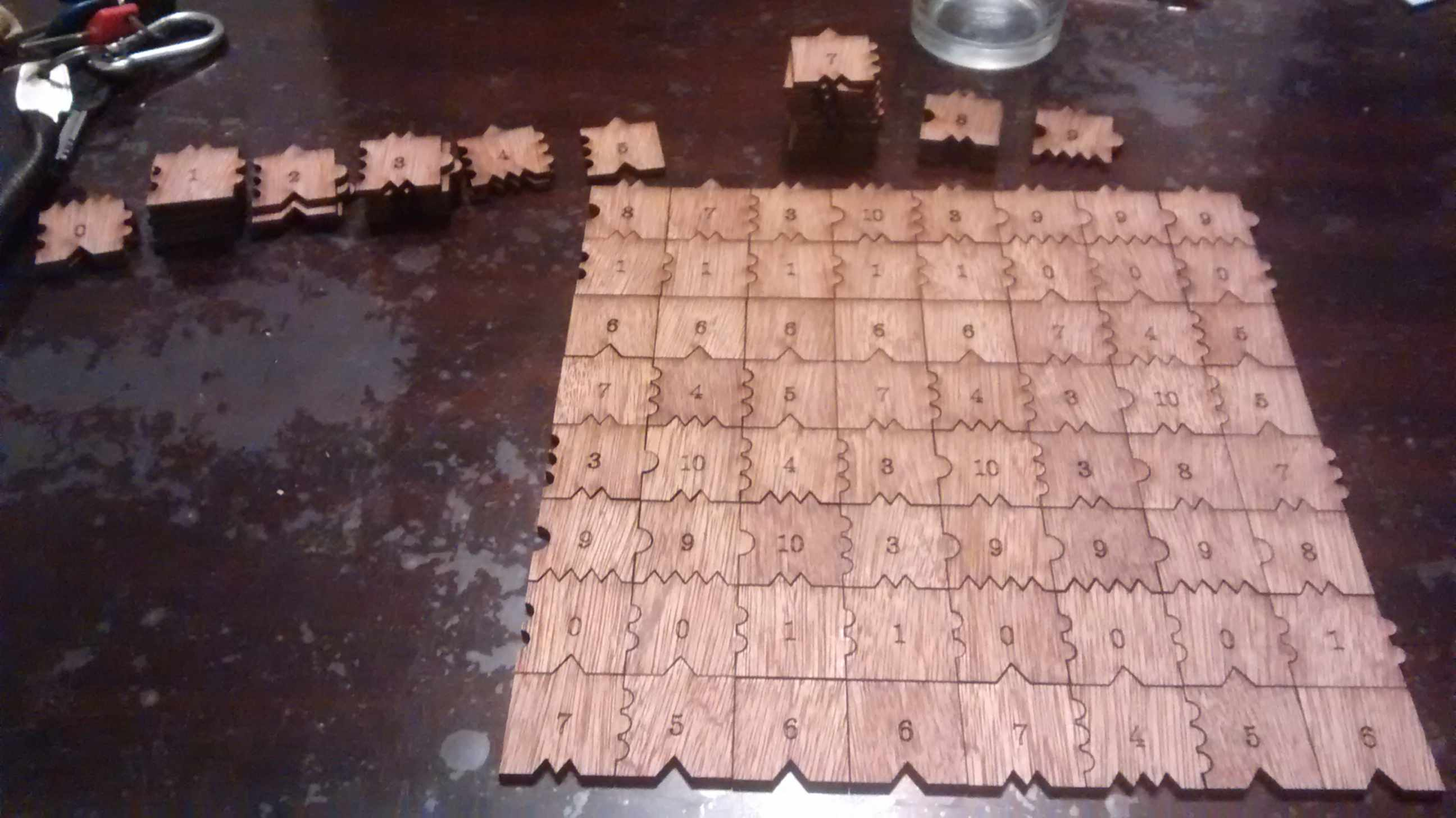One may recreate the 33 x 19 tiling as follows (note that I am using Cartesian-like coordinates, so the first list data actually is the first column from bottom to top):

sage: data = [[10, 4, 6, 1, 3, 3, 7, 0, 9, 7, 2, 6, 1, 3, 8, 7, 0, 9, 7],
....:  [4, 5, 6, 1, 8, 10, 4, 0, 9, 3, 8, 7, 0, 9, 7, 5, 0, 9, 3],
....:  [3, 7, 6, 1, 7, 2, 5, 0, 9, 8, 7, 5, 0, 9, 3, 7, 0, 9, 10],
....:  [10, 4, 6, 1, 3, 8, 7, 0, 9, 7, 5, 6, 1, 8, 10, 4, 0, 9, 3],
....:  [2, 5, 6, 1, 8, 7, 5, 0, 9, 3, 7, 6, 1, 7, 2, 5, 0, 9, 8],
....:  [8, 7, 6, 1, 7, 5, 6, 1, 8, 10, 4, 6, 1, 3, 8, 7, 0, 9, 7],
....:  [7, 5, 6, 1, 3, 7, 6, 1, 7, 2, 5, 6, 1, 8, 7, 5, 0, 9, 3],
....:  [3, 7, 6, 1, 10, 4, 6, 1, 3, 8, 7, 6, 1, 7, 5, 6, 1, 8, 10],
....:  [10, 4, 6, 1, 3, 3, 7, 0, 9, 7, 5, 6, 1, 3, 7, 6, 1, 7, 2],
....:  [2, 5, 6, 1, 8, 10, 4, 0, 9, 3, 7, 6, 1, 10, 4, 6, 1, 3, 8],
....:  [8, 7, 6, 1, 7, 5, 5, 0, 9, 10, 4, 6, 1, 3, 3, 7, 0, 9, 7],
....:  [7, 5, 6, 1, 3, 7, 6, 1, 10, 4, 5, 6, 1, 8, 10, 4, 0, 9, 3],
....:  [3, 7, 6, 1, 10, 4, 6, 1, 3, 3, 7, 6, 1, 7, 2, 5, 0, 9, 8],
....:  [10, 4, 6, 1, 3, 3, 7, 0, 9, 10, 4, 6, 1, 3, 8, 7, 0, 9, 7],
....:  [4, 5, 6, 1, 8, 10, 4, 0, 9, 3, 3, 7, 0, 9, 7, 5, 0, 9, 3],
....:  [3, 7, 6, 1, 7, 2, 5, 0, 9, 8, 10, 4, 0, 9, 3, 7, 0, 9, 10],
....:  [10, 4, 6, 1, 3, 8, 7, 0, 9, 7, 5, 5, 0, 9, 10, 4, 0, 9, 3],
....:  [2, 5, 6, 1, 8, 7, 5, 0, 9, 3, 7, 6, 1, 10, 4, 5, 0, 9, 8],
....:  [8, 7, 6, 1, 7, 5, 6, 1, 8, 10, 4, 6, 1, 3, 3, 7, 0, 9, 7],
....:  [7, 5, 6, 1, 3, 7, 6, 1, 7, 2, 5, 6, 1, 8, 10, 4, 0, 9, 3],
....:  [3, 7, 6, 1, 10, 4, 6, 1, 3, 8, 7, 6, 1, 7, 2, 5, 0, 9, 8],
....:  [10, 4, 6, 1, 3, 3, 7, 0, 9, 7, 2, 6, 1, 3, 8, 7, 0, 9, 7],
....:  [4, 5, 6, 1, 8, 10, 4, 0, 9, 3, 8, 7, 0, 9, 7, 5, 0, 9, 3],
....:  [3, 7, 6, 1, 7, 2, 5, 0, 9, 8, 7, 5, 0, 9, 3, 7, 0, 9, 10],
....:  [10, 4, 6, 1, 3, 8, 7, 0, 9, 7, 5, 6, 1, 8, 10, 4, 0, 9, 3],
....:  [3, 3, 7, 0, 9, 7, 5, 0, 9, 3, 7, 6, 1, 7, 2, 5, 0, 9, 8],
....:  [8, 10, 4, 0, 9, 3, 7, 0, 9, 10, 4, 6, 1, 3, 8, 7, 0, 9, 7],
....:  [7, 5, 5, 0, 9, 10, 4, 0, 9, 3, 3, 7, 0, 9, 7, 5, 0, 9, 3],
....:  [3, 7, 6, 1, 10, 4, 5, 0, 9, 8, 10, 4, 0, 9, 3, 7, 0, 9, 10],
....:  [10, 4, 6, 1, 3, 3, 7, 0, 9, 7, 5, 5, 0, 9, 10, 4, 0, 9, 3],
....:  [2, 5, 6, 1, 8, 10, 4, 0, 9, 3, 7, 6, 1, 10, 4, 5, 0, 9, 8],
....:  [8, 7, 6, 1, 7, 5, 5, 0, 9, 10, 4, 6, 1, 3, 3, 7, 0, 9, 7],
....:  [7, 5, 6, 1, 3, 7, 6, 1, 10, 4, 5, 6, 1, 8, 10, 4, 0, 9, 3]]

The above patch have been chosen among 1000 other randomly generated as the closest to the asymptotic frequencies of the tiles in Jeandel-Rao tilings (or at least in the minimal subshift that I describe in the preprint):

sage: from collections import Counter
sage: c = Counter(flatten(data))
sage: tile_count = [c[i] for i in range(11)]

The asymptotic frequencies:

sage: phi = golden_ratio.n()
sage: Linv = [2*phi + 6, 2*phi + 6, 18*phi + 10, 2*phi + 6, 8*phi + 2,
....:      5*phi + 4, 2*phi + 6, 12/5*phi + 14/5, 8*phi + 2,
....:      2*phi + 6, 8*phi + 2]
sage: perfect_proportions = vector([1/a for a in Linv])

Comparison of the number of tiles of each type with the expected frequency:

sage: header_row = ['tile id', 'Asymptotic frequency', 'Expected nb of copies',
....:               'Nb copies in the 33x19 patch']
sage: columns = [range(11), perfect_proportions, vector(perfect_proportions)*33*19, tile_count]
tile id   Asymptotic frequency   Expected nb of copies   Nb copies in the 33x19 patch
+---------+----------------------+-----------------------+------------------------------+
0         0.108271182329550      67.8860313206280        67
1         0.108271182329550      67.8860313206280        65
2         0.0255593590340479     16.0257181143480        16
3         0.108271182329550      67.8860313206280        71
4         0.0669152706817991     41.9558747174880        42
5         0.0827118232955023     51.8603132062800        51
6         0.108271182329550      67.8860313206280        65
7         0.149627093977301      93.8161879237680        95
8         0.0669152706817991     41.9558747174880        44
9         0.108271182329550      67.8860313206280        67
10        0.0669152706817991     41.9558747174880        44

I brought the $$33\times19=641$$ tiles at the conference and offered to the first 7 persons to find a $$7\times 7$$ tiling the opportunity to keep the 49 tiles they used. 49 is a good number since the frequency of the lowest tile (with id 2) is about 2% which allows to have at least one copy of each tile in a subset of 49 tiles allowing a solution.

A natural question to ask is how many such $$7\times 7$$ tilings does there exist? With ticket #25125 that was merged in Sage 8.3 this Spring, it is possible to enumerate and count solutions in parallel with Knuth dancing links algorithm. After the installation of the Sage Optional package slabbe (sage -pip install slabbe), one may compute that there are 152244 solutions.

sage: from slabbe import WangTileSet
sage: tiles = [(2,4,2,1), (2,2,2,0), (1,1,3,1), (1,2,3,2), (3,1,3,3),
....: (0,1,3,1), (0,0,0,1), (3,1,0,2), (0,2,1,2), (1,2,1,4), (3,3,1,2)]
sage: T0 = WangTileSet(tiles)
sage: T0_solver = T0.solver(7,7)
sage: %time T0_solver.number_of_solutions(ncpus=8)
CPU times: user 16 ms, sys: 82.3 ms, total: 98.3 ms
Wall time: 388 ms
152244

One may also get the list of all solutions and print one of them:

sage: %time L = T0_solver.all_solutions(); print(len(L))
152244
CPU times: user 6.46 s, sys: 344 ms, total: 6.8 s
Wall time: 6.82 s
sage: L
A wang tiling of a 7 x 7 rectangle
sage: L.table()  # warning: the output is in Cartesian-like coordinates
[[1, 8, 10, 4, 5, 0, 9],
[1, 7, 2, 5, 6, 1, 8],
[1, 3, 8, 7, 6, 1, 7],
[0, 9, 7, 5, 6, 1, 3],
[0, 9, 3, 7, 6, 1, 8],
[1, 8, 10, 4, 6, 1, 7],
[1, 7, 2, 2, 6, 1, 3]]

This is the number of distinct sets of 49 tiles which admits a 7x7 solution:

sage: from collections import Counter
sage: def count_tiles(tiling):
....:     C = Counter(flatten(tiling.table()))
....:     return tuple(C.get(a,0) for a in range(11))
sage: Lfreq = map(count_tiles, L)
sage: Lfreq_count = Counter(Lfreq)
sage: len(Lfreq_count)
83258

Number of other solutions with the same set of 49 tiles:

sage: Counter(Lfreq_count.values())
Counter({1: 49076, 2: 19849, 3: 6313, 4: 3664, 6: 1410, 5: 1341, 7: 705, 8:
293, 9: 159, 14: 116, 10: 104, 12: 97, 18: 44, 11: 26, 15: 24, 13: 10, 17: 8,
22: 6, 32: 6, 16: 3, 28: 2, 19: 1, 21: 1})

How the number of $$k\times k$$-solutions grows for k from 0 to 9:

sage: [T0.solver(k,k).number_of_solutions() for k in range(10)]
[0, 11, 85, 444, 1723, 9172, 50638, 152244, 262019, 1641695]

Unfortunately, most of those $$k\times k$$-solutions are not extendable to a tiling of the whole plane. Indeed the number of $$k\times k$$ patches in the language of the minimal aperiodic subshift that I am able to describe and which is a proper subset of Jeandel-Rao tilings seems, according to some heuristic, to be something like:

[1, 11, 49, 108, 184, 268, 367, 483]

I do not share my (ugly) code for this computation yet, as I will rather share clean code soon when times come. So among the 152244 about only 483 (0.32%) of them are prolongable into a uniformly recurrent tiling of the plane.

]]>
Sébastien Labbé http://www.slabbe.org/blogue <![CDATA[A time evolution picture of packages built in parallel by Sage]]> http://www.slabbe.org/blogue/2016/12/a-time-evolution-picture-of-packages-built-in-parallel-by-sage 2016-12-16T16:13:00Z 2016-12-16T16:13:00Z

Compiling sage takes a while and does a lot of stuff. Each time I am wondering which components takes so much time and which are fast. I wrote a module in my slabbe version 0.3b2 package available on PyPI to figure this out.

This is after compiling 7.5.beta6 after an upgrade from 7.5.beta4:

sage: from slabbe.analyze_sage_build import draw_sage_build
sage: draw_sage_build().pdf()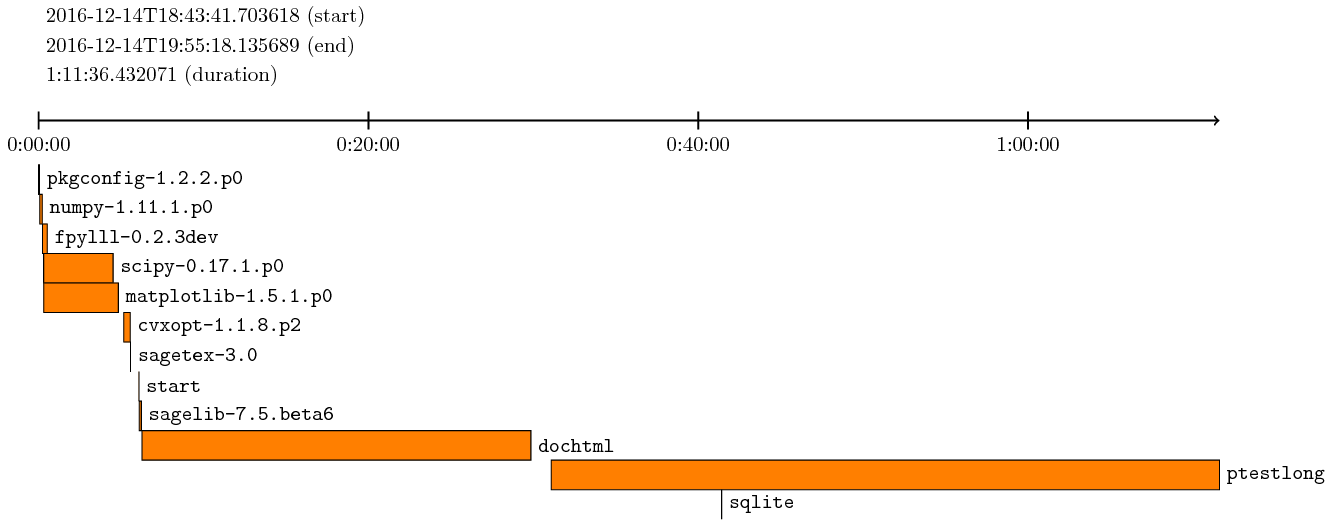From scratch from a fresh git clone of 7.5.beta6, after running MAKE='make -j4' make ptestlong, I get:

sage: from slabbe.analyze_sage_build import draw_sage_build
sage: draw_sage_build().pdf()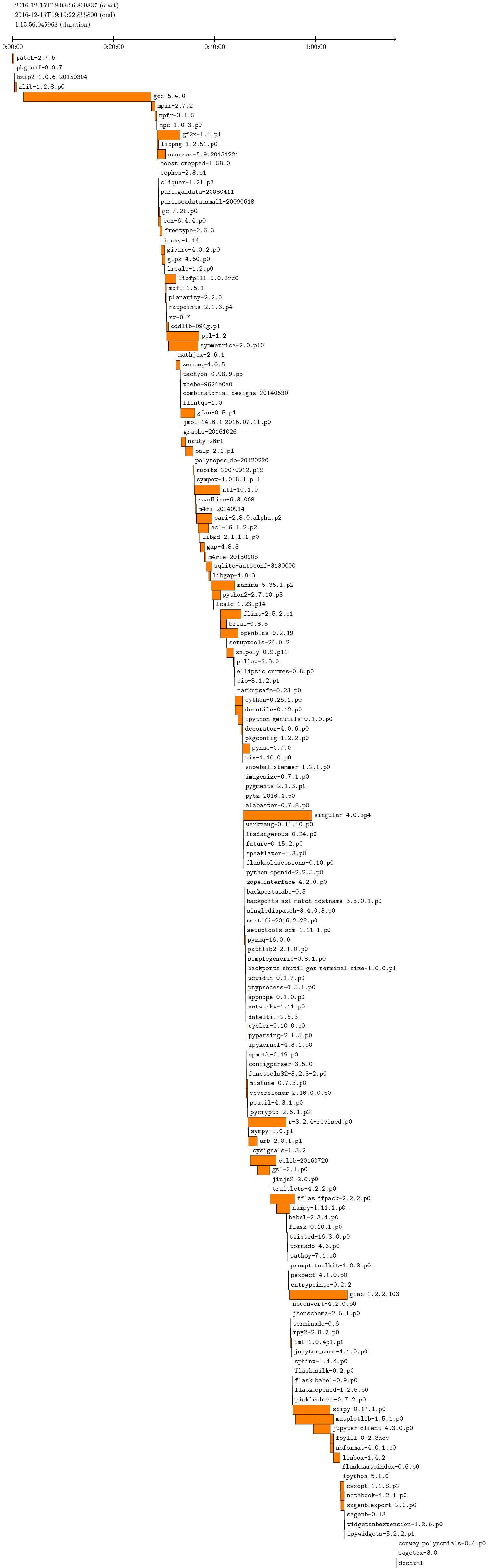The picture does not include the start and ptestlong because there was an error compiling the documentation.

By default, draw_sage_build considers all of the logs files in logs/pkgs but options are available to consider only log files created in a given interval of time. See draw_sage_build? for more info.

]]>
Sébastien Labbé http://www.slabbe.org/blogue <![CDATA[unsupported operand parent for *, Matrix over number field, vector over symbolic ring]]> http://www.slabbe.org/blogue/2016/02/unsupported-operand-parent-for-matrix-over-number-field-vector-over-symbolic-ring 2016-02-18T10:17:00Z 2016-02-18T10:17:00Z

Yesterday I received this email (in french):

Salut,
avec Thomas on a une question bête:

K.<x>=NumberField(x*x-x-1)

J'aimerais multiplier une matrice avec des coefficients en x par un vecteur
contenant des variables a et b.  Il dit "unsupported operand parent for *,
Matrix over number field, vector over symbolic ring"

Est ce grave ?

Here is my answer. Indeed, in Sage, symbolic variables can't multiply with elements in an Number Field in x:

sage: x = var('x')
sage: K.<x> = NumberField(x*x-x-1)
sage: a = var('a')
sage: a*x
Traceback (most recent call last)
...
TypeError: unsupported operand parent(s) for '*': 'Symbolic Ring' and
'Number Field in x with defining polynomial x^2 - x - 1'

But, we can define a polynomial ring with variables in a,b and coefficients in the NumberField. Then, we are able to multiply a with x:

sage: x = var('x')
sage: K.<x> = NumberField(x*x-x-1)
sage: K
Number Field in x with defining polynomial x^2 - x - 1
sage: R.<a,b> = K['a','b']
sage: R
Multivariate Polynomial Ring in a, b over Number Field in x with
defining polynomial x^2 - x - 1
sage: a*x
(x)*a

With two square brackets, we obtain powers series:

sage: R.<a,b> = K[['a','b']]
sage: R
Multivariate Power Series Ring in a, b over Number Field in x with
defining polynomial x^2 - x - 1
sage: a*x*b
(x)*a*b

It works with matrices:

sage: MS = MatrixSpace(R,2,2)
sage: MS
Full MatrixSpace of 2 by 2 dense matrices over Multivariate Power
Series Ring in a, b over Number Field in x with defining polynomial
x^2 - x - 1
sage: MS([0,a,b,x])
[  0   a]
[  b (x)]
sage: m1 = MS([0,a,b,x])
sage: m2 = MS([0,a+x,b*b+x,x*x])
sage: m1 + m2 * m1
[              (x)*b + a*b       (x + 1) + (x + 1)*a]
[                (x + 2)*b (3*x + 1) + (x)*a + a*b^2]
]]>
Sébastien Labbé http://www.slabbe.org/blogue <![CDATA[slabbe-0.2.spkg released]]> http://www.slabbe.org/blogue/2015/11/slabbe-0.2.spkg-released 2015-11-30T11:53:00Z 2015-11-30T11:53:00Z

These is a summary of the functionalities present in slabbe-0.2.spkg optional Sage package. It works on version 6.8 of Sage but will work best with sage-6.10 (it is using the new code for cartesian_product merged the the betas of sage-6.10). It contains 7 new modules:

• finite_word.py
• language.py
• lyapunov.py
• matrix_cocycle.py
• mult_cont_frac.pyx
• ranking_scale.py
• tikz_picture.py

Cheat Sheets

The best way to have a quick look at what can be computed with the optional Sage package slabbe-0.2.spkg is to look at the 3-dimensional Continued Fraction Algorithms Cheat Sheets available on the arXiv since today. It gathers a handful of informations on different 3-dimensional Continued Fraction Algorithms including well-known and old ones (Poincaré, Brun, Selmer, Fully Subtractive) and new ones (Arnoux-Rauzy-Poincaré, Reverse, Cassaigne).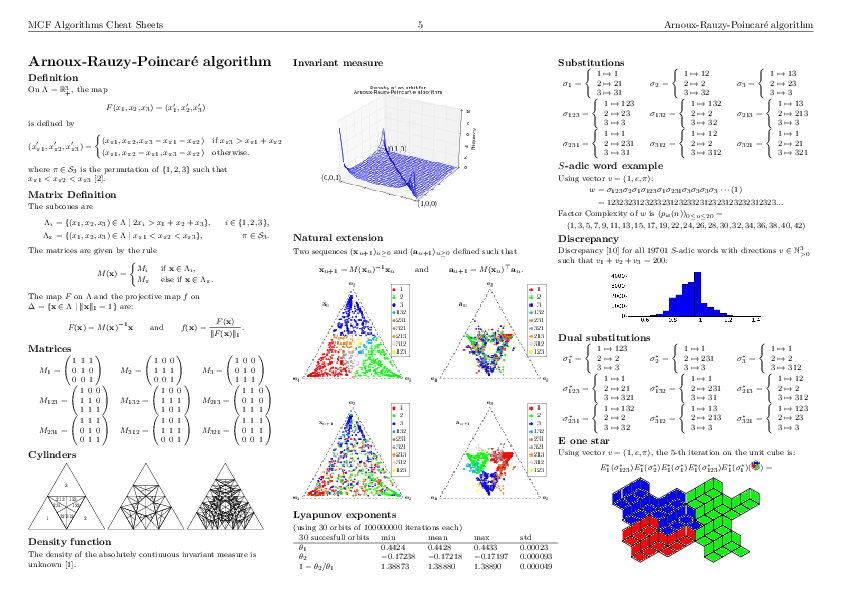Installation

sage -i http://www.slabbe.org/Sage/slabbe-0.2.spkg    # on sage 6.8
sage -p http://www.slabbe.org/Sage/slabbe-0.2.spkg    # on sage 6.9 or beyond

Examples

Computing the orbit of Brun algorithm on some input in $$\mathbb{R}^3_+$$ including dual coordinates:

sage: from slabbe.mult_cont_frac import Brun
sage: algo = Brun()
sage: algo.cone_orbit_list((100, 87, 15), 4)
[(13.0, 87.0, 15.0, 1.0, 2.0, 1.0, 321),
(13.0, 72.0, 15.0, 1.0, 2.0, 3.0, 132),
(13.0, 57.0, 15.0, 1.0, 2.0, 5.0, 132),
(13.0, 42.0, 15.0, 1.0, 2.0, 7.0, 132)]

Computing the invariant measure:

sage: fig = algo.invariant_measure_wireframe_plot(n_iterations=10^6, ndivs=30)
sage: fig.savefig('a.png')Drawing the cylinders:

sage: cocycle = algo.matrix_cocycle()
sage: t = cocycle.tikz_n_cylinders(3, scale=3)
sage: t.png()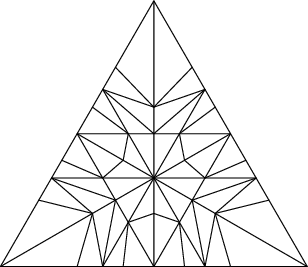Computing the Lyapunov exponents of the 3-dimensional Brun algorithm:

sage: from slabbe.lyapunov import lyapunov_table
sage: lyapunov_table(algo, n_orbits=30, n_iterations=10^7)
30 succesful orbits     min       mean      max       std
+-----------------------+---------+---------+---------+---------+
$\theta_1$              0.3026    0.3045    0.3051    0.00046
$\theta_2$              -0.1125   -0.1122   -0.1115   0.00020
$1-\theta_2/\theta_1$   1.3680    1.3684    1.3689    0.00024

Dealing with tikzpictures

Since I create lots of tikzpictures in my code and also because I was unhappy at how the view command of Sage handles them (a tikzpicture is not a math expression to put inside dollar signs), I decided to create a class for tikzpictures. I think this module could be useful in Sage so I will propose its inclusion soon.

I am using the standalone document class which allows some configurations like the border:

sage: from slabbe import TikzPicture
sage: g = graphs.PetersenGraph()
sage: s = latex(g)
sage: t = TikzPicture(s, standalone_configs=["border=4mm"], packages=['tkz-graph'])

The repr method does not print all of the string since it is often very long. Though it shows how many lines are not printed:

sage: t
\documentclass[tikz]{standalone}
\standaloneconfig{border=4mm}
\usepackage{tkz-graph}
\begin{document}
\begin{tikzpicture}
%
\useasboundingbox (0,0) rectangle (5.0cm,5.0cm);
%
\definecolor{cv0}{rgb}{0.0,0.0,0.0}
...
... 68 lines not printed (3748 characters in total) ...
...
\Edge[lw=0.1cm,style={color=cv6v8,},](v6)(v8)
\Edge[lw=0.1cm,style={color=cv6v9,},](v6)(v9)
\Edge[lw=0.1cm,style={color=cv7v9,},](v7)(v9)
%
\end{tikzpicture}
\end{document}

There is a method to generates a pdf and another for generating a png. Both opens the file in a viewer by default unless view=False:

sage: pathtofile = t.png(density=60, view=False)
sage: pathtofile = t.pdf()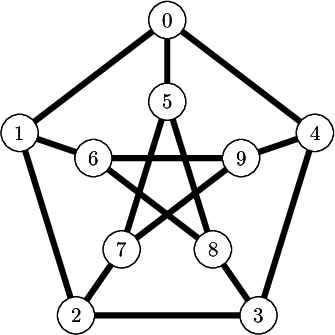Compare this with the output of view(s, tightpage=True) which does not allow to control the border and also creates a second empty page on some operating system (osx, only one page on ubuntu):

sage: view(s, tightpage=True)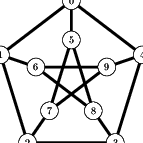One can also provide the filename where to save the file in which case the file is not open in a viewer:

sage: _ = t.pdf('petersen_graph.pdf')

Another example with polyhedron code taken from this Sage thematic tutorial Draw polytopes in LateX using TikZ:

sage: V = [[1,0,1],[1,0,0],[1,1,0],[0,0,-1],[0,1,0],[-1,0,0],[0,1,1],[0,0,1],[0,-1,0]]
sage: P = Polyhedron(vertices=V).polar()
sage: s = P.projection().tikz([674,108,-731],112)
sage: t = TikzPicture(s)
sage: t
\documentclass[tikz]{standalone}
\begin{document}
\begin{tikzpicture}%
[x={(0.249656cm, -0.577639cm)},
y={(0.777700cm, -0.358578cm)},
z={(-0.576936cm, -0.733318cm)},
scale=2.000000,
...
... 80 lines not printed (4889 characters in total) ...
...
\node[vertex] at (1.00000, 1.00000, -1.00000)     {};
\node[vertex] at (1.00000, 1.00000, 1.00000)     {};
%%
%%
\end{tikzpicture}
\end{document}
sage: _ = t.pdf()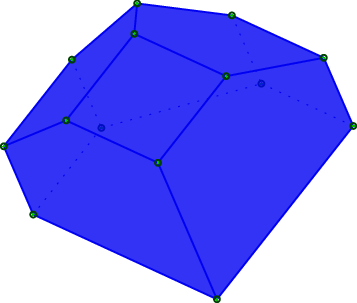]]>
Sébastien Labbé http://www.slabbe.org/blogue <![CDATA[There are 13.366.431.646 solutions to the Quantumino game]]> http://www.slabbe.org/blogue/2015/09/there-are-13.366.431.646-solutions-to-the-quantumino-game 2015-09-21T14:55:00Z 2015-09-21T14:55:00Z

Some years ago, I wrote code in Sage to solve the Quantumino puzzle. I also used it to make a one-minute video illustrating the Dancing links algorithm which I am proud to say it is now part of the Dancing links wikipedia page.Let me recall that the goal of the Quantumino puzzle is to fill a $$2\times 5\times 8$$ box with 16 out of 17 three-dimensional pentaminos. After writing the sage code to solve the puzzle, one question was left: how many solutions are there? Is the official website realist or very prudent when they say that there are over 10.000 potential solutions? Can it be computed in hours? days? months? years? The only thing I knew was that the following computation (letting the 0-th pentamino aside) never finished on my machine:

sage: from sage.games.quantumino import QuantuminoSolver
sage: QuantuminoSolver(0).number_of_solutions()   # long time :)

Since I spent already too much time on this side-project, I decided in 2012 to stop investing any more time on it and to really focus on finishing writing my thesis.

So before I finish writing my thesis, I knew that the computation was not going to take a light-year, since I was able to finish the computation of the number of solutions when the 0-th pentamino is put aside and when one pentamino is pre-positioned somewhere in the box. That computation completed in 4 hours on my old laptop and gave about 5 millions solutions. There are 17 choices of pentatminos to put aside, there are 360 distinct positions of that pentamino in the box, so I estimated the number of solution to be something like $$17\times 360\times 5000000 = 30 \times 10^9$$. Most importantly, I estimated the computation to take $$17\times 360\times 4= 24480$$ hours or 1020 days. Therefore, I knew I could not do it on my laptop.

But last year, I received an email from the designer of the Quantumino puzzle:

-------- Message transféré --------
Sujet : quantumino
Date : Tue, 09 Dec 2014 13:22:30 +0100
De : Nicolaas Neuwahl
Pour : Sebastien Labbe

hi sébastien labbé,

i'm the designer of the quantumino puzzle.
i'm not a mathematician, i'm an architect. i like mathematics.
i'm quite impressed to see the sage work on quantumino, also i have not the
knowledge for full understanding.

i have a question for you - can you tell me HOW MANY different quantumino-
solutions exist?

ty and bye

nicolaas neuwahl

This summer was a good timing to launch the computation on my beautiful Intel® Core™ i5-4590 CPU @ 3.30GHz × 4 at Université de Liège. First, I improved the Sage code to allow a parallel computation of number of solutions in the dancing links code (#18987, merged in a Sage 6.9.beta6). Secondly, we may remark that each tiling of the $$2\times 5\times 8$$ box can be rotated in order to find 3 other solutions. It is possible to gain a factor 4 by avoiding to count 4 times the same solution up to rotations (#19107, still needs work from myself). Thanks to Vincent Delecroix for doing the review on both ticket. Dividing the estimated 1024 days of computation needed by a factor $$4\times 4=16$$ gives an approximation of 64 days to complete the computation. Two months, just enough to be tractable!

With those two tickets (some previous version to be honest) on top of sage-6.8, I started the computation on August 4th and the computation finished last week on September 18th for a total of 45 days. The computation was stopped only once on September 8th (I forgot to close firefox and thunderbird that night...).

The number of solutions and computation time for each pentamino put aside together with the first solution found is shown in the table below. We remark that some values are equal when the aside pentaminoes are miror images (why!?:).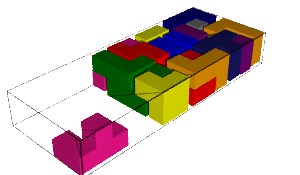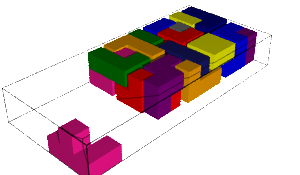634 900 493 solutions 634 900 493 solutions 2 days, 6:22:44.883358 2 days, 6:19:08.945691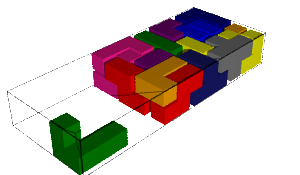509 560 697 solutions 509 560 697 solutions 2 days, 0:01:36.844612 2 days, 0:41:59.447773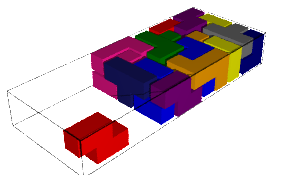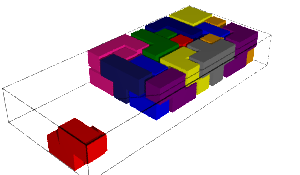628 384 422 solutions 628 384 422 solutions 2 days, 7:52:31.459247 2 days, 8:44:49.465672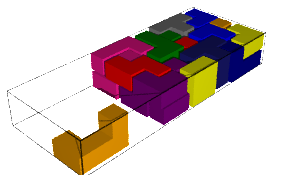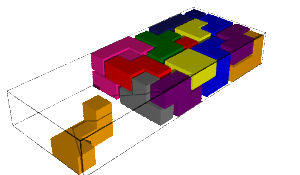1 212 362 145 solutions 1 212 362 145 solutions 3 days, 17:25:00.346627 3 days, 19:10:02.353063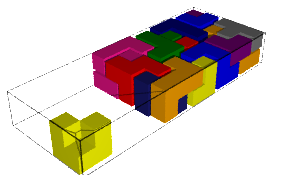197 325 298 solutions 556 534 800 solutions 22:51:54.439932 1 day, 19:05:23.908326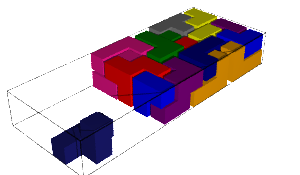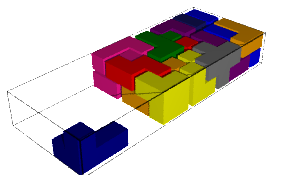664 820 756 solutions 468 206 736 solutions 2 days, 8:48:54.767662 1 day, 20:14:56.014557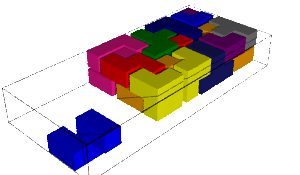1 385 955 043 solutions 1 385 955 043 solutions 4 days, 1:40:30.270929 4 days, 4:44:05.399367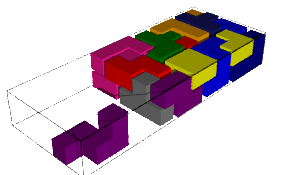694 998 374 solutions 694 998 374 solutions 2 days, 11:44:29.631 2 days, 6:01:57.946708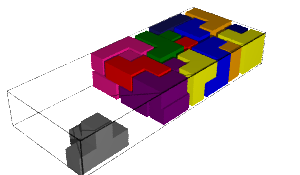1 347 221 708 solutions 3 days, 21:51:29.043459

Therefore the total number of solutions up to rotations is 13 366 431 646 which is indeed more than 10000:)

sage: L = [634900493, 634900493, 509560697, 509560697, 628384422,
628384422, 1212362145, 1212362145, 197325298, 556534800, 664820756,
468206736, 1385955043, 1385955043, 694998374, 694998374, 1347221708]
sage: sum(L)
13366431646
sage: factor(_)
2 * 23 * 271 * 1072231
 The machine (4 cores) Intel® Core™ i5-4590 CPU @ 3.30GHz × 4 (Université de Liège) Computation Time 45 days, (Aug 4th -- Sep 18th, 2015) Number of solutions (up to rotations) 13 366 431 646 Number of solutions / cpu / second 859

My code will be available on github.

About the video on wikipedia.

I must say that the video is not perfect. On wikipedia, the file talk page of the video says that the Jerky camera movement is distracting. That is because I managed to make the video out of images created by .show(viewer='tachyon') which changes the coordinate system, hardcodes a lot of parameters, zoom properly, simplifies stuff to make sure the user don't see just a blank image. But, for making a movie, we need access to more parameters especially the placement of the camera (to avoid the jerky movement). I know that Tachyon allows all of that. It is still a project that I have to create a more versatile Graphics3D -> Tachyon conversion allowing to construct nice videos of evolving mathematical objects. That's another story.

]]>
Sébastien Labbé http://www.slabbe.org/blogue <![CDATA[Arnoux-Rauzy-Poincaré sequences]]> http://www.slabbe.org/blogue/2015/02/arnoux-rauzy-poincare-sequences 2015-02-26T16:22:00Z 2015-02-26T16:22:00Z

In a recent article with Valérie Berthé [BL15], we provided a multidimensional continued fraction algorithm called Arnoux-Rauzy-Poincaré (ARP) to construct, given any vector $$v\in\mathbb{R}_+^3$$, an infinite word $$w\in\{1,2,3\}^\mathbb{N}$$ over a three-letter alphabet such that the frequencies of letters in $$w$$ exists and are equal to $$v$$ and such that the number of factors (i.e. finite block of consecutive letters) of length $$n$$ appearing in $$w$$ is linear and less than $$\frac{5}{2}n+1$$. We also conjecture that for almost all $$v$$ the contructed word describes a discrete path in the positive octant staying at a bounded distance from the euclidean line of direction $$v$$.

In Sage, you can construct this word using the next version of my package slabbe-0.2 (not released yet, email me to press me to finish it). The one with frequencies of letters proportionnal to $$(1, e, \pi)$$ is:

sage: from slabbe.mcf import algo
sage: D = algo.arp.substitutions()
sage: it = algo.arp.coding_iterator((1,e,pi))
sage: w = words.s_adic(it, repeat(1), D)
word: 1232323123233231232332312323123232312323...

The factor complexity is close to 2n+1 and the balance is often less or equal to three:

sage: w[:10000].number_of_factors(100)
202
sage: w[:100000].number_of_factors(1000)
2002
sage: w[:1000].balance()
3
sage: w[:2000].balance()
3

Note that bounded distance from the euclidean line almost surely was proven in [DHS2013] for Brun algorithm, another MCF algorithm.

Other approaches: Standard model and billiard sequences

Other approaches have been proposed to construct such discrete lines.

One of them is the standard model of Eric Andres [A03]. It is also equivalent to billiard sequences in the cube. It is well known that the factor complexity of billiard sequences is quadratic $$p(n)=n^2+n+1$$ [AMST94]. Experimentally, we can verify this. We first create a billiard word of some given direction:

sage: from slabbe import BilliardCube
sage: v = vector(RR, (1, e, pi))
sage: b = BilliardCube(v)
sage: b
Cubic billiard of direction (1.00000000000000, 2.71828182845905, 3.14159265358979)
sage: w = b.to_word()
sage: w
word: 3231232323123233213232321323231233232132...

We create some prefixes of $$w$$ that we represent internally as char*. The creation is slow because the implementation of billiard words in my optional package is in Python and is not that efficient:

sage: p3 = Word(w[:10^3], alphabet=[1,2,3], datatype='char')
sage: p4 = Word(w[:10^4], alphabet=[1,2,3], datatype='char') # takes 3s
sage: p5 = Word(w[:10^5], alphabet=[1,2,3], datatype='char') # takes 32s
sage: p6 = Word(w[:10^6], alphabet=[1,2,3], datatype='char') # takes 5min 20s

We see below that exactly $$n^2+n+1$$ factors of length $$n<20$$ appears in the prefix of length 1000000 of $$w$$:

sage: A = ['n'] + range(30)
sage: c3 = ['p_(w[:10^3])(n)'] + map(p3.number_of_factors, range(30))
sage: c4 = ['p_(w[:10^4])(n)'] + map(p4.number_of_factors, range(30))
sage: c5 = ['p_(w[:10^5])(n)'] + map(p5.number_of_factors, range(30)) # takes 4s
sage: c6 = ['p_(w[:10^6])(n)'] + map(p6.number_of_factors, range(30)) # takes 49s
sage: ref = ['n^2+n+1'] + [n^2+n+1 for n in range(30)]
sage: T = table(columns=[A,c3,c4,c5,c6,ref])
sage: T
n    p_(w[:10^3])(n)   p_(w[:10^4])(n)   p_(w[:10^5])(n)   p_(w[:10^6])(n)   n^2+n+1
+----+-----------------+-----------------+-----------------+-----------------+---------+
0    1                 1                 1                 1                 1
1    3                 3                 3                 3                 3
2    7                 7                 7                 7                 7
3    13                13                13                13                13
4    21                21                21                21                21
5    31                31                31                31                31
6    43                43                43                43                43
7    52                55                56                57                57
8    63                69                71                73                73
9    74                85                88                91                91
10   87                103               107               111               111
11   100               123               128               133               133
12   115               145               151               157               157
13   130               169               176               183               183
14   144               195               203               211               211
15   160               223               232               241               241
16   176               253               263               273               273
17   192               285               296               307               307
18   208               319               331               343               343
19   224               355               368               381               381
20   239               392               407               421               421
21   254               430               448               463               463
22   268               470               491               507               507
23   282               510               536               553               553
24   296               552               583               601               601
25   310               596               632               651               651
26   324               642               683               703               703
27   335               687               734               757               757
28   345               734               787               813               813
29   355               783               842               871               871

Billiard sequences generate paths that are at a bounded distance from an euclidean line. This is equivalent to say that the balance is finite. The balance is defined as the supremum value of difference of the number of apparition of a letter in two factors of the same length. For billiard sequences, the balance is 2:

sage: p3.balance()
2
sage: p4.balance() # takes 2min 37s
2

Other approaches: Melançon and Reutenauer

Melançon and Reutenauer [MR13] also suggested a method that generalizes Christoffel words in higher dimension. The construction is based on the application of two substitutions generalizing the construction of sturmian sequences. Below we compute the factor complexity and the balance of some of their words over a three-letter alphabet.

On a three-letter alphabet, the two morphisms are:

sage: L = WordMorphism('1->1,2->13,3->2')
sage: R = WordMorphism('1->13,2->2,3->3')
sage: L
WordMorphism: 1->1, 2->13, 3->2
sage: R
WordMorphism: 1->13, 2->2, 3->3

Example 1: periodic case $$LRLRLRLRLR\dots$$. In this example, the factor complexity seems to be around $$p(n)=2.76n$$ and the balance is at least 28:

sage: from itertools import repeat, cycle
sage: W = words.s_adic(cycle((L,R)),repeat('1'))
sage: W
word: 1213122121313121312212212131221213131213...
sage: map(W[:10000].number_of_factors, [10,20,40,80])
[27, 54, 110, 221]
sage: [27/10., 54/20., 110/40., 221/80.]
[2.70000000000000, 2.70000000000000, 2.75000000000000, 2.76250000000000]
sage: W[:1000].balance()  # takes 1.6s
21
sage: W[:2000].balance()  # takes 6.4s
28

Example 2: $$RLR^2LR^4LR^8LR^{16}LR^{32}LR^{64}LR^{128}\dots$$ taken from the conclusion of their article. In this example, the factor complexity seems to be $$p(n)=3n$$ and balance at least as high (=bad) as $$122$$:

sage: W = words.s_adic([R,L,R,R,L,R,R,R,R,L]+[R]*8+[L]+[R]*16+[L]+[R]*32+[L]+[R]*64+[L]+[R]*128,'1')
sage: W.length()
330312
sage: map(W.number_of_factors, [10, 20, 100, 200, 300, 1000])
[29, 57, 295, 595, 895, 2981]
sage: [29/10., 57/20., 295/100., 595/200., 895/300., 2981/1000.]
[2.90000000000000,
2.85000000000000,
2.95000000000000,
2.97500000000000,
2.98333333333333,
2.98100000000000]
sage: W[:1000].balance()  # takes 1.6s
122
sage: W[:2000].balance()  # takes 6s
122

Example 3: some random ones. The complexity $$p(n)/n$$ occillates between 2 and 3 for factors of length $$n=1000$$ in prefixes of length 100000:

sage: for _ in range(10):
....:     W = words.s_adic([choice((L,R)) for _ in range(50)],'1')
....:     print W[:100000].number_of_factors(1000)/1000.
2.02700000000000
2.23600000000000
2.74000000000000
2.21500000000000
2.78700000000000
2.52700000000000
2.85700000000000
2.33300000000000
2.65500000000000
2.51800000000000

For ten randomly generated words, the balance goes from 6 to 27 which is much more than what is obtained for billiard words or by our approach:

sage: for _ in range(10):
....:     W = words.s_adic([choice((L,R)) for _ in range(50)],'1')
....:     print W[:1000].balance(), W[:2000].balance()
12 15
8 24
14 14
5 11
17 17
14 14
6 6
19 27
9 16
12 12
]]>
Sébastien Labbé http://www.slabbe.org/blogue <![CDATA[Abelian complexity of the Oldenburger sequence]]> http://www.slabbe.org/blogue/2014/09/abelian-complexity-of-the-oldenburger-sequence 2014-09-27T22:00:00Z 2014-09-27T22:00:00Z

The Oldenburger infinite sequence [O39] $K = 1221121221221121122121121221121121221221\ldots$ also known under the name of Kolakoski, is equal to its exponent trajectory. The exponent trajectory $$\Delta$$ can be obtained by counting the lengths of blocks of consecutive and equal letters: $K = 1^12^21^22^11^12^21^12^21^22^11^22^21^12^11^22^11^12^21^22^11^22^11^12^21^12^21^22^11^12^21^12^11^22^11^22^21^12^21^2\ldots$ The sequence of exponents above gives the exponent trajectory of the Oldenburger sequence: $\Delta = 12211212212211211221211212\ldots$ which is equal to the original sequence $$K$$. You can define this sequence in Sage:

sage: K = words.KolakoskiWord()
sage: K
word: 1221121221221121122121121221121121221221...
sage: K.delta()          # delta returns the exponent trajectory
word: 1221121221221121122121121221121121221221...

There are a lot of open problem related to basic properties of that sequence. For example, we do not know if that sequence is recurrent, that is, all finite subword or factor (finite block of consecutive letters) always reappear. Also, it is still open to prove whether the density of 1 in that sequence is equal to $$1/2$$.

In this blog post, I do some computations on its abelian complexity $$p_{ab}(n)$$ defined as the number of distinct abelian vectors of subwords of length $$n$$ in the sequence. The abelian vector $$\vec{w}$$ of a word $$w$$ counts the number of occurences of each letter: $w = 12211212212 \quad \mapsto \quad 1^5 2^7 \text{, abelianized} \quad \mapsto \quad \vec{w} = (5, 7) \text{, the abelian vector of } w$

Here are the abelian vectors of subwords of length 10 and 20 in the prefix of length 100 of the Oldenburger sequence. The functions abelian_vectors and abelian_complexity are not in Sage as of now. Code is available at trac #17058 to be merged in Sage soon:

sage: prefix = words.KolakoskiWord()[:100]
sage: prefix.abelian_vectors(10)
{(4, 6), (5, 5), (6, 4)}
sage: prefix.abelian_vectors(20)
{(8, 12), (9, 11), (10, 10), (11, 9), (12, 8)}

Therefore, the prefix of length 100 has 3 vectors of subwords of length 10 and 5 vectors of subwords of length 20:

sage: p100.abelian_complexity(10)
3
sage: p100.abelian_complexity(20)
5

I import the OldenburgerSequence from my optional spkg because it is faster than the implementation in Sage:

sage: from slabbe import KolakoskiWord as OldenburgerSequence
sage: Olden = OldenburgerSequence()

I count the number of abelian vectors of subwords of length 100 in the prefix of length $$2^{20}$$ of the Oldenburger sequence:

sage: prefix = Olden[:2^20]
sage: %time prefix.abelian_vectors(100)
CPU times: user 3.48 s, sys: 66.9 ms, total: 3.54 s
Wall time: 3.56 s
{(47, 53), (48, 52), (49, 51), (50, 50), (51, 49), (52, 48), (53, 47)}

Number of abelian vectors of subwords of length less than 100 in the prefix of length $$2^{20}$$ of the Oldenburger sequence:

sage: %time L100 = map(prefix.abelian_complexity, range(100))
CPU times: user 3min 20s, sys: 1.08 s, total: 3min 21s
Wall time: 3min 23s
sage: from collections import Counter
sage: Counter(L100)
Counter({5: 26, 6: 26, 4: 17, 7: 15, 3: 8, 8: 4, 2: 3, 1: 1})

Let's draw that:

sage: labels = ('Length of factors', 'Number of abelian vectors')
sage: title = 'Abelian Complexity of the prefix of length $2^{20}$ of Oldenburger sequence'
sage: list_plot(L100, color='green', plotjoined=True, axes_labels=labels, title=title)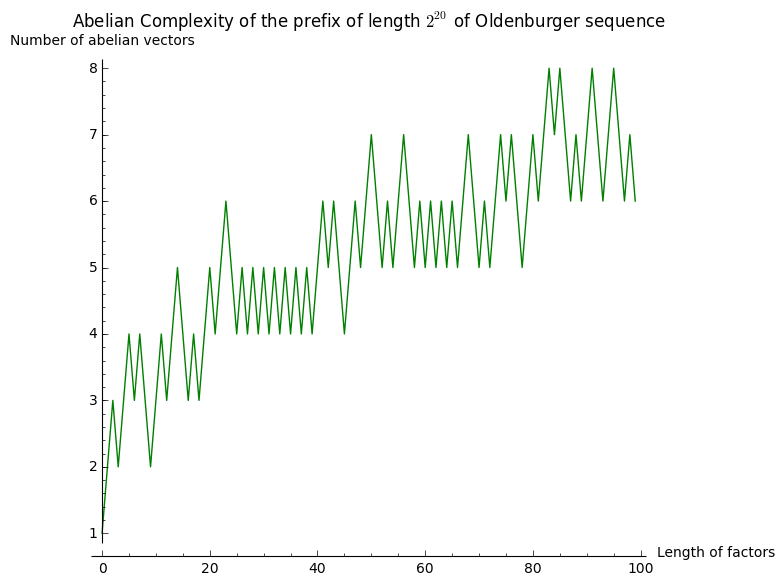It seems to grow something like $$\log(n)$$. Let's now consider subwords of length $$2^n$$ for $$0\leq n\leq 12$$ in the same prefix of length $$2^{20}$$:

sage: %time L20 = [(2^n, prefix.abelian_complexity(2^n)) for n in range(20)]
CPU times: user 41 s, sys: 239 ms, total: 41.2 s
Wall time: 41.5 s
sage: L20
[(1, 2), (2, 3), (4, 3), (8, 3), (16, 3), (32, 5), (64, 5), (128, 9),
(256, 9), (512, 13), (1024, 17), (2048, 22), (4096, 27), (8192, 40),
(16384, 46), (32768, 67), (65536, 81), (131072, 85), (262144, 90), (524288, 104)]

I now look at subwords of length $$2^n$$ for $$0\leq n\leq 23$$ in the longer prefix of length $$2^{24}$$:

sage: prefix = Olden[:2^24]
sage: %time L24 = [(2^n, prefix.abelian_complexity(2^n)) for n in range(24)]
CPU times: user 20min 47s, sys: 13.5 s, total: 21min
Wall time: 20min 13s
sage: L24
[(1, 2), (2, 3), (4, 3), (8, 3), (16, 3), (32, 5), (64, 5), (128, 9), (256,
9), (512, 13), (1024, 17), (2048, 23), (4096, 33), (8192, 46), (16384, 58),
(32768, 74), (65536, 98), (131072, 134), (262144, 165), (524288, 229),
(1048576, 302), (2097152, 371), (4194304, 304), (8388608, 329)]

The next graph gather all of the above computations:

sage: G = Graphics()
sage: legend = 'in the prefix of length 2^{}'
sage: G += list_plot(L24, plotjoined=True, thickness=4, color='blue', legend_label=legend.format(24))
sage: G += list_plot(L20, plotjoined=True, thickness=4, color='red', legend_label=legend.format(20))
sage: G += list_plot(L100, plotjoined=True, thickness=4, color='green', legend_label=legend.format(20))
sage: labels = ('Length of factors', 'Number of abelian vectors')
sage: title = 'Abelian complexity of Oldenburger sequence'
sage: G.show(scale=('semilogx', 2), axes_labels=labels, title=title)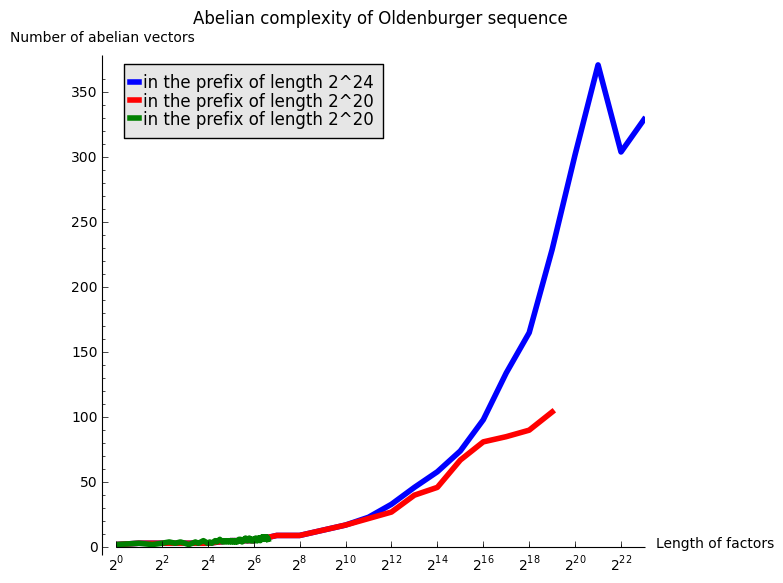A linear growth in the above graphics with logarithmic $$x$$ abcisse would mean a growth in $$\log(n)$$. After those experimentations, my hypothesis is that the abelian complexity of the Oldenburger sequence grows like $$\log(n)^2$$.

References

 [O39] Oldenburger, Rufus (1939). "Exponent trajectories in symbolic dynamics". Transactions of the American Mathematical Society 46: 453–466. doi:10.2307/1989933
]]>
Sébastien Labbé http://www.slabbe.org/blogue <![CDATA[slabbe-0.1.spkg released]]> http://www.slabbe.org/blogue/2014/08/slabbe-0.1.spkg-released 2014-08-27T16:53:00Z 2014-08-27T16:53:00Z

These is a summary of the functionalities present in slabbe-0.1 optional Sage package. It depends on version 6.3 of Sage because it uses RecursivelyEnumeratedSet code that was merged in 6.3. It contains modules on digital geometry, combinatorics on words and more.

Install the optional spkg (depends on sage-6.3):

sage -i http://www.liafa.univ-paris-diderot.fr/~labbe/Sage/slabbe-0.1.spkg

In each of the example below, you first have to import the module once and for all:

sage: from slabbe import *

To construct the image below, make sure to use tikz package so that view is able to compile tikz code when called:

sage: latex.extra_preamble()
'\\usepackage{tikz}'

Draw the part of a discrete plane

sage: p = DiscretePlane([1,pi,7], 1+pi+7, mu=0)
sage: d = DiscreteTube([-5,5],[-5,5])
sage: I = p & d
sage: I
Intersection of the following objects:
Set of points x in ZZ^3 satisfying: 0 <= (1, pi, 7) . x + 0 < pi + 8
DiscreteTube: Preimage of [-5, 5] x [-5, 5] by a 2 by 3 matrix
sage: clip = d.clip()
sage: tikz = I.tikz(clip=clip)
sage: view(tikz, tightpage=True)Draw the part of a discrete line

sage: L = DiscreteLine([-2,3], 5)
sage: b = DiscreteBox([0,10], [0,10])
sage: I = L & b
sage: I
Intersection of the following objects:
Set of points x in ZZ^2 satisfying: 0 <= (-2, 3) . x + 0 < 5
[0, 10] x [0, 10]
sage: I.plot()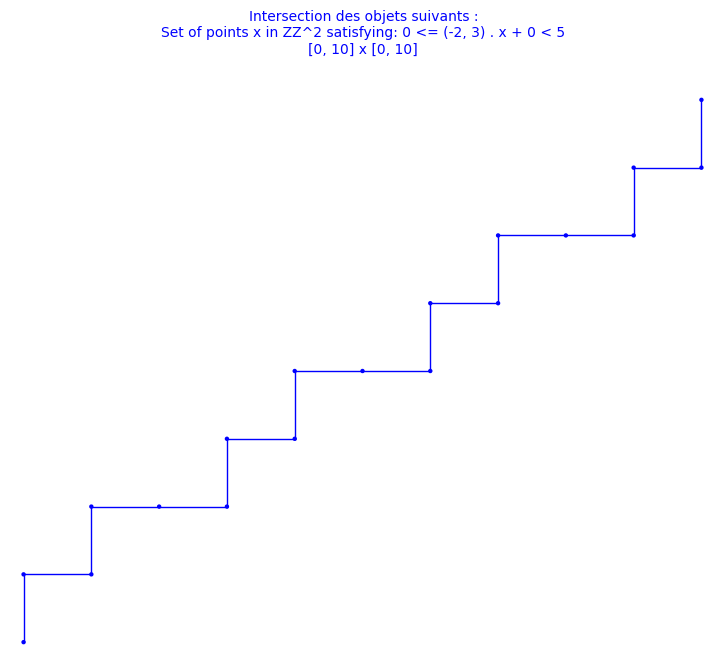Double square tiles

This module was developped for the article on the combinatorial properties of double square tiles written with Ariane Garon and Alexandre Blondin Massé [BGL2012]. The original version of the code was written with Alexandre.

sage: D = DoubleSquare((34,21,34,21))
sage: D
Double Square Tile
w0 = 3032321232303010303230301012101030   w4 = 1210103010121232121012123230323212
w1 = 323030103032321232303                w5 = 101212321210103010121
w2 = 2321210121232303232123230301030323   w6 = 0103032303010121010301012123212101
w3 = 212323032321210121232                w7 = 030101210103032303010
(|w0|, |w1|, |w2|, |w3|) = (34, 21, 34, 21)
(d0, d1, d2, d3)         = (42, 68, 42, 68)
(n0, n1, n2, n3)         = (0, 0, 0, 0)
sage: D.plot()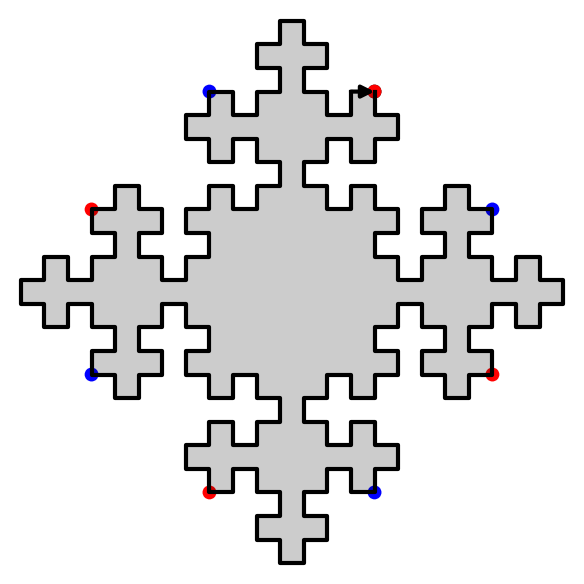sage: D.extend(0).extend(1).plot()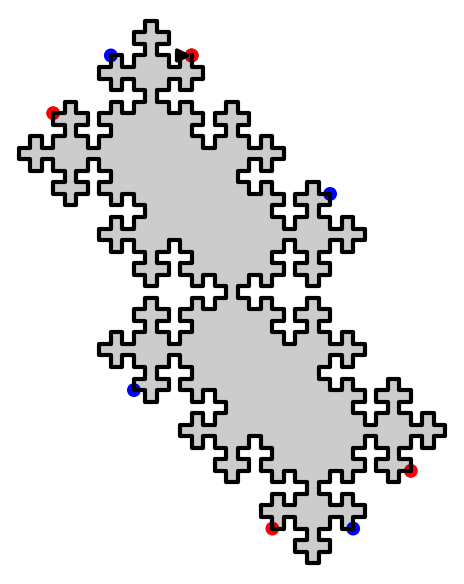We have shown that using two invertible operations (called SWAP and TRIM), every double square tiles can be reduced to the unit square:

sage: D.plot_reduction()The reduction operations are:

sage: D.reduction()
['SWAP_1', 'TRIM_1', 'TRIM_3', 'SWAP_1', 'TRIM_1', 'TRIM_3', 'TRIM_0', 'TRIM_2']

The result of the reduction is the unit square:

sage: unit_square = D.apply(D.reduction())
sage: unit_square
Double Square Tile
w0 =     w4 =
w1 = 3   w5 = 1
w2 =     w6 =
w3 = 2   w7 = 0
(|w0|, |w1|, |w2|, |w3|) = (0, 1, 0, 1)
(d0, d1, d2, d3)         = (2, 0, 2, 0)
(n0, n1, n2, n3)         = (0, NaN, 0, NaN)
sage: unit_square.plot()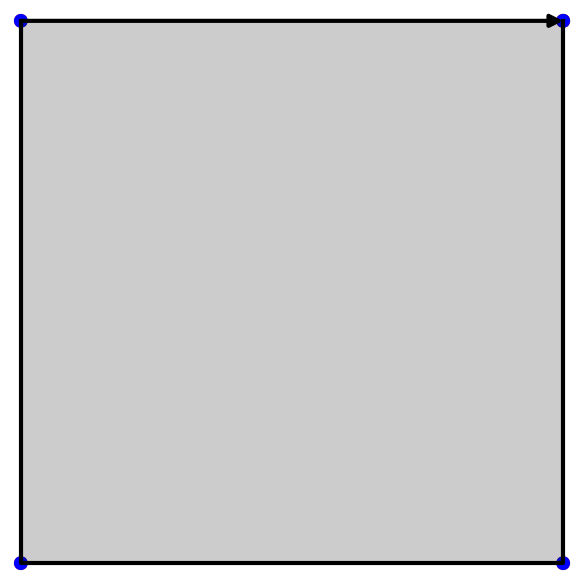Since SWAP and TRIM are invertible operations, we can recover every double square from the unit square:

sage: E = unit_square.extend(2).extend(0).extend(3).extend(1).swap(1).extend(3).extend(1).swap(1)
sage: D == E
True

Christoffel graphs

This module was developped for the article on a d-dimensional extension of Christoffel Words written with Christophe Reutenauer [LR2014].

sage: G = ChristoffelGraph((6,10,15))
sage: G
Christoffel set of edges for normal vector v=(6, 10, 15)
sage: tikz = G.tikz_kernel()
sage: view(tikz, tightpage=True)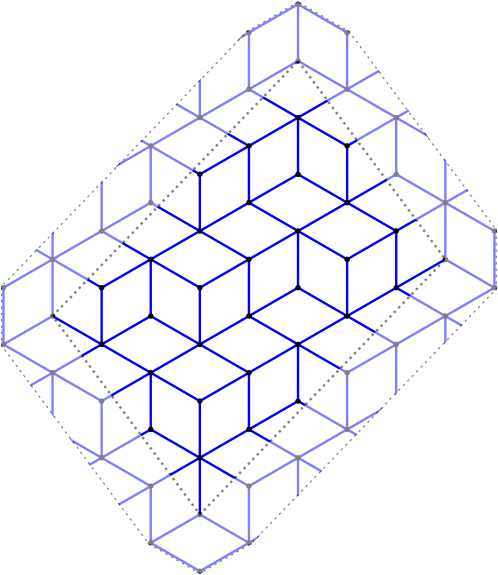Bispecial extension types

This module was developped for the article on the factor complexity of infinite sequences genereated by substitutions written with Valérie Berthé [BL2014].

The extension type of an ordinary bispecial factor:

sage: L = [(1,3), (2,3), (3,1), (3,2), (3,3)]
sage: E = ExtensionType1to1(L, alphabet=(1,2,3))
sage: E
E(w)   1   2   3
1             X
2             X
3     X   X   X
m(w)=0, ordinary
sage: E.is_ordinaire()
True

Creation of a strong-weak pair of bispecial words from a neutral not ordinaire word:

sage: p23 = WordMorphism({1:[1,2,3],2:[2,3],3:})
sage: e = ExtensionType1to1([(1,2),(2,3),(3,1),(3,2),(3,3)], [1,2,3])
sage: e
E(w)   1   2   3
1         X
2             X
3     X   X   X
m(w)=0, not ord.
sage: A,B = e.apply(p23)
sage: A
E(3w)   1   2   3
1
2         X   X
3     X   X   X
m(w)=1, not ord.
sage: B
E(23w)   1   2   3
1          X
2
3              X
m(w)=-1, not ord.

Fast Kolakoski word

This module was written for fun. It uses cython implementation inspired from the 10 lines of C code written by Dominique Bernardi and shared during Sage Days 28 in Orsay, France, in January 2011.

sage: K = KolakoskiWord()
sage: K
word: 1221121221221121122121121221121121221221...
sage: %time K[10^5]
CPU times: user 1.56 ms, sys: 7 µs, total: 1.57 ms
Wall time: 1.57 ms
1
sage: %time K[10^6]
CPU times: user 15.8 ms, sys: 30 µs, total: 15.8 ms
Wall time: 15.9 ms
2
sage: %time K[10^8]
CPU times: user 1.58 s, sys: 2.28 ms, total: 1.58 s
Wall time: 1.59 s
1
sage: %time K[10^9]
CPU times: user 15.8 s, sys: 12.4 ms, total: 15.9 s
Wall time: 15.9 s
1

This is much faster than the Python implementation available in Sage:

sage: K = words.KolakoskiWord()
sage: %time K[10^5]
CPU times: user 779 ms, sys: 25.9 ms, total: 805 ms
Wall time: 802 ms
1

References

 [BGL2012] A. Blondin Massé, A. Garon, S. Labbé, Combinatorial properties of double square tiles, Theoretical Computer Science 502 (2013) 98-117. doi:10.1016/j.tcs.2012.10.040
 [LR2014] Labbé, Sébastien, and Christophe Reutenauer. A d-dimensional Extension of Christoffel Words. arXiv:1404.4021 (April 15, 2014).
 [BL2014] V. Berthé, S. Labbé, Factor Complexity of S-adic sequences generated by the Arnoux-Rauzy-Poincaré Algorithm. arXiv:1404.4189 (April, 2014).
]]>
Sébastien Labbé http://www.slabbe.org/blogue <![CDATA[Releasing slabbe, my own Sage package]]> http://www.slabbe.org/blogue/2014/08/releasing-slabbe-my-own-sage-package 2014-08-27T16:48:00Z 2014-08-27T16:48:00Z

Since two years I wrote thousands of line of private code for my own research. Each module having between 500 and 2000 lines of code. The code which is the more clean corresponds to code written in conjunction with research articles. People who know me know that I systematically put docstrings and doctests in my code to facilitate reuse of the code by myself, but also in the idea of sharing it and eventually making it public.

I did not made that code into Sage because it was not mature enough. Also, when I tried to make a complete module go into Sage (see #13069 and #13346), then the monstrous never evolving #12224 became a dependency of the first and the second was unofficially reviewed asking me to split it into smaller chunks to make the review process easier. I never did it because I spent already too much time on it (making a module 100% doctested takes time). Also, the module was corresponding to a published article and I wanted to leave it just like that.

Getting new modules into Sage is hard

In general, the introduction of a complete new module into Sage is hard especially for beginners. Here are two examples I feel responsible for: #10519 is 4 years old and counting, the author has a new work and responsabilities; in #12996, the author was decouraged by the amount of work given by the reviewers. There is a lot of things a beginner has to consider to obtain a positive review. And even for a more advanced developper, other difficulties arise. Indeed, a module introduces a lot of new functions and it may also introduce a lot of new bugs... and Sage developpers are sometimes reluctant to give it a positive review. And if it finally gets a positive review, it is not available easily to normal users of Sage until the next release of Sage.

Releasing my own Sage package

Still I felt the need around me to make my code public. But how? There are people (a few of course but I know there are) who are interested in reproducing computations and images done in my articles. This is why I came to the idea of releasing my own Sage package containing my public research code. This way both developpers and colleagues that are user of Sage but not developpers will be able to install and use my code. This will make people more aware if there is something useful in a module for them. And if one day, somebody tells me: "this should be in Sage", then I will be able to say : "I agree! Do you want to review it?".

Old style Sage package vs New sytle git Sage package

Then I had to chose between the old and the new style for Sage packages. I did not like the new style, because

• I wanted the history of my package to be independant of the history of Sage,
• I wanted it to be as easy to install as sage -i slabbe,
• I wanted it to work on any recent enough version of Sage,
• I wanted to be able to release a new version, give it to a colleague who could install it right away without changing its own Sage (i.e., updating the checksums).

Therefore, I choose the old style. I based my work on other optional Sage packages, namely the SageManifolds spkg and the ore_algebra spkg.

Content of the initial version

The initial version of the slabbe Sage package has modules concerning four topics: Digital geometry, Combinatorics on words, Combinatorics and Python class inheritance.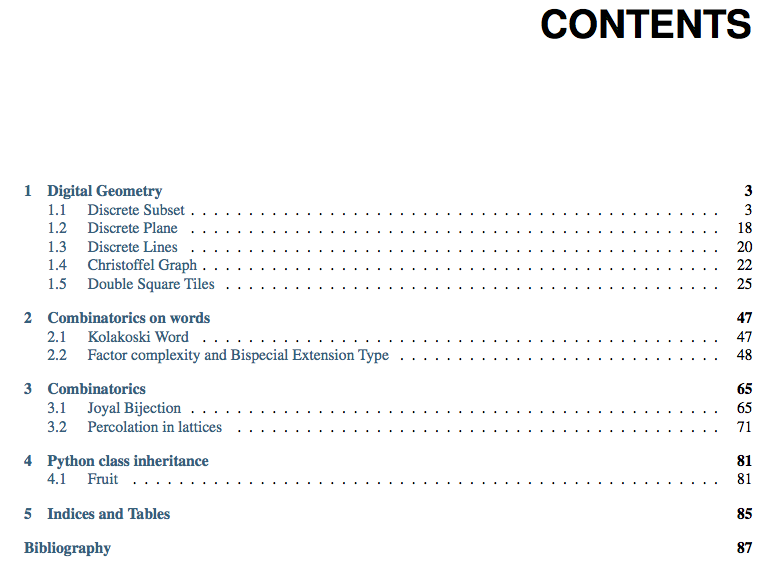For installation or for release notes of the initial version of the spkg, consult the slabbe spkg section of the Sage page of this website.

]]>
Sébastien Labbé http://www.slabbe.org/blogue <![CDATA[My status report at Sage Days 57 (RecursivelyEnumeratedSet)]]> http://www.slabbe.org/blogue/2014/04/my-status-report-at-sage-days-57-recursivelyenumeratedset 2014-04-11T17:15:00Z 2014-04-11T17:15:00Z

At Sage Days 57, I worked on the trac ticket #6637: standardize the interface to TransitiveIdeal and friends. My patch proposes to replace TransitiveIdeal and SearchForest by a new class called RecursivelyEnumeratedSet that would handle every case.

A set S is called recursively enumerable if there is an algorithm that enumerates the members of S. We consider here the recursively enumerated set that are described by some seeds and a successor function succ. The successor function may have some structure (symmetric, graded, forest) or not. Many kinds of iterators are provided: depth first search, breadth first search or elements of given depth.

Consider the permutations of $$\{1,2,3\}$$ and the poset generated by the method permutohedron_succ:

sage: P = Permutations(3)
sage: d = {p:p.permutohedron_succ() for p in P}
sage: S = Poset(d)
sage: S.plot()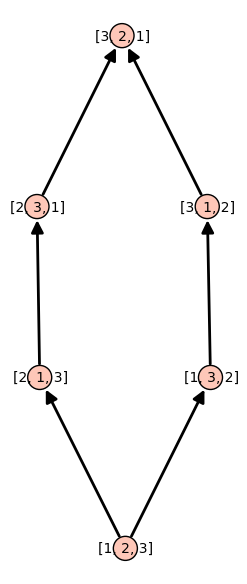The TransitiveIdeal allows to generates all permutations from the identity permutation using the method permutohedron_succ as successor function:

sage: succ = attrcall("permutohedron_succ")
sage: seed = [Permutation([1,2,3])]
sage: T = TransitiveIdeal(succ, seed)
sage: list(T)
[[1, 2, 3], [2, 1, 3], [1, 3, 2], [2, 3, 1], [3, 2, 1], [3, 1, 2]]

Remark that the previous ordering is neither breadth first neither depth first. It is a naive search because it stores the element to process in a set instead of a queue or a stack.

Note that the method permutohedron_succ produces a graded poset. Therefore, one may use the TransitiveIdealGraded class instead:

sage: T = TransitiveIdealGraded(succ, seed)
sage: list(T)
[[1, 2, 3], [2, 1, 3], [1, 3, 2], [2, 3, 1], [3, 1, 2], [3, 2, 1]]

For TransitiveIdealGraded, the enumeration is breadth first search. Althougth, if you look at the code (version Sage 6.1.1 or earlier), we see that this iterator do not make use of the graded hypothesis at all because the known set remembers every generated elements:

current_level = self._generators
known = set(current_level)
depth = 0
while len(current_level) > 0 and depth <= self._max_depth:
next_level = set()
for x in current_level:
yield x
for y in self._succ(x):
if y == None or y in known:
continue
current_level = next_level
depth += 1
return

Timings for TransitiveIdeal

sage: succ = attrcall("permutohedron_succ")
sage: seed = [Permutation([1..5])]
sage: T = TransitiveIdeal(succ, seed)
sage: %time L = list(T)
CPU times: user 26.6 ms, sys: 1.57 ms, total: 28.2 ms
Wall time: 28.5 ms
sage: seed = [Permutation([1..8])]
sage: T = TransitiveIdeal(succ, seed)
sage: %time L = list(T)
CPU times: user 14.4 s, sys: 141 ms, total: 14.5 s
Wall time: 14.8 s

sage: seed = [Permutation([1..5])]
sage: T = TransitiveIdealGraded(succ, seed)
sage: %time L = list(T)
CPU times: user 25.3 ms, sys: 1.04 ms, total: 26.4 ms
Wall time: 27.4 ms
sage: seed = [Permutation([1..8])]
sage: T = TransitiveIdealGraded(succ, seed)
sage: %time L = list(T)
CPU times: user 14.5 s, sys: 85.8 ms, total: 14.5 s
Wall time: 14.7 s

In conlusion, use TransitiveIdeal for naive search algorithm and use TransitiveIdealGraded for breadth search algorithm. Both class do not use the graded hypothesis.

Recursively enumerated set with a graded structure

The new class RecursivelyEnumeratedSet provides all iterators for each case. The example below are for the graded case.

Depth first search iterator:

sage: succ = attrcall("permutohedron_succ")
sage: seed = [Permutation([1..5])]
sage: R = RecursivelyEnumeratedSet(seed, succ, structure='graded')
sage: it_depth = R.depth_first_search_iterator()
sage: [next(it_depth) for _ in range(5)]
[[1, 2, 3, 4, 5],
[1, 2, 3, 5, 4],
[1, 2, 5, 3, 4],
[1, 2, 5, 4, 3],
[1, 5, 2, 4, 3]]

Breadth first search iterator:

sage: [next(it_breadth) for _ in range(5)]
[[1, 2, 3, 4, 5],
[1, 3, 2, 4, 5],
[1, 2, 4, 3, 5],
[2, 1, 3, 4, 5],
[1, 2, 3, 5, 4]]

Elements of given depth iterator:

sage: list(R.elements_of_depth_iterator(9))
[[5, 4, 2, 3, 1], [4, 5, 3, 2, 1], [5, 3, 4, 2, 1], [5, 4, 3, 1, 2]]
sage: list(R.elements_of_depth_iterator(10))
[[5, 4, 3, 2, 1]]

Levels (a level is a set of elements of the same depth):

sage: R.level(0)
[[1, 2, 3, 4, 5]]
sage: R.level(1)
{[1, 2, 3, 5, 4], [1, 2, 4, 3, 5], [1, 3, 2, 4, 5], [2, 1, 3, 4, 5]}
sage: R.level(2)
{[1, 2, 4, 5, 3],
[1, 2, 5, 3, 4],
[1, 3, 2, 5, 4],
[1, 3, 4, 2, 5],
[1, 4, 2, 3, 5],
[2, 1, 3, 5, 4],
[2, 1, 4, 3, 5],
[2, 3, 1, 4, 5],
[3, 1, 2, 4, 5]}
sage: R.level(3)
{[1, 2, 5, 4, 3],
[1, 3, 4, 5, 2],
[1, 3, 5, 2, 4],
[1, 4, 2, 5, 3],
[1, 4, 3, 2, 5],
[1, 5, 2, 3, 4],
[2, 1, 4, 5, 3],
[2, 1, 5, 3, 4],
[2, 3, 1, 5, 4],
[2, 3, 4, 1, 5],
[2, 4, 1, 3, 5],
[3, 1, 2, 5, 4],
[3, 1, 4, 2, 5],
[3, 2, 1, 4, 5],
[4, 1, 2, 3, 5]}
sage: R.level(9)
{[4, 5, 3, 2, 1], [5, 3, 4, 2, 1], [5, 4, 2, 3, 1], [5, 4, 3, 1, 2]}
sage: R.level(10)
{[5, 4, 3, 2, 1]}

Recursively enumerated set with a symmetric structure

We construct a recursively enumerated set with symmetric structure and depth first search for default enumeration algorithm:

sage: succ = lambda a: [(a-1,a), (a,a-1), (a+1,a), (a,a+1)]
sage: seeds = [(0,0)]
sage: C = RecursivelyEnumeratedSet(seeds, succ, structure='symmetric', algorithm='depth')
sage: C
A recursively enumerated set with a symmetric structure (depth first search)

In this case, depth first search is the default algorithm for iteration:

sage: it_depth = iter(C)
sage: [next(it_depth) for _ in range(10)]
[(0, 0), (0, 1), (0, 2), (0, 3), (0, 4), (0, 5), (0, 6), (0, 7), (0, 8), (0, 9)]

Breadth first search. This algorithm makes use of the symmetric structure and remembers only the last two levels:

sage: [next(it_breadth) for _ in range(10)]
[(0, 0), (0, 1), (0, -1), (1, 0), (-1, 0), (-1, 1), (-2, 0), (0, 2), (2, 0), (-1, -1)]

Levels (elements of given depth):

sage: sorted(C.level(0))
[(0, 0)]
sage: sorted(C.level(1))
[(-1, 0), (0, -1), (0, 1), (1, 0)]
sage: sorted(C.level(2))
[(-2, 0), (-1, -1), (-1, 1), (0, -2), (0, 2), (1, -1), (1, 1), (2, 0)]

Timings for RecursivelyEnumeratedSet

We get same timings as for TransitiveIdeal but it uses less memory so it might be able to enumerate bigger sets:

sage: succ = attrcall("permutohedron_succ")
sage: seed = [Permutation([1..5])]
sage: R = RecursivelyEnumeratedSet(seed, succ, structure='graded')
sage: %time L = list(R)
CPU times: user 24.7 ms, sys: 1.33 ms, total: 26.1 ms
Wall time: 26.4 ms
sage: seed = [Permutation([1..8])]
sage: R = RecursivelyEnumeratedSet(seed, succ, structure='graded')
sage: %time L = list(R)
CPU times: user 14.5 s, sys: 70.2 ms, total: 14.5 s
Wall time: 14.6 s
]]>
Sébastien Labbé http://www.slabbe.org/blogue <![CDATA[Demo of the IPython notebook at Sage Paris group meeting]]> http://www.slabbe.org/blogue/2014/03/demo-of-the-ipython-notebook-at-sage-paris-group-meeting 2014-03-06T10:47:00Z 2014-03-06T10:47:00Z

Today I am presenting the IPython notebook at the meeting of the Sage Paris group. This post gathers what I prepared.

Installation

First you can install the ipython notebook in Sage as explained in this previous blog post. If everything works, then you run:

sage -ipython notebook

and this will open a browser.

Turn on Sage preparsing

Create a new notebook and type:

In : 3 + 3
6
In : 2 / 3
0
In : matrix
Traceback (most recent call last):
...
NameError: name 'matrix' is not defined

By default, Sage preparsing is turn off and Sage commands are not known. To turn on the Sage preparsing (thanks to a post of Jason on sage-devel):

Since sage-6.2, according to sage-devel, the command is:

You now get Sage commands working in ipython:

In : 3 + 4
Out: 7
In : 2 / 3
Out: 2/3
In : type(_)
Out: <type 'sage.rings.rational.Rational'>
In : matrix(3, range(9))
Out:
[0 1 2]
[3 4 5]
[6 7 8]

Scroll and hide output

If the output is too big, click on Out to scroll or hide the output:

In : range(1000)

Sage 3d Graphics

3D graphics works but open in a new Jmol window:

In : sphere()

Sage 2d Graphics

Similarly, 2D graphics works but open in a new window:

In : plot(sin(x), (x,0,10))

Inline Matplotlib graphics

To create inline matplotlib graphics, the notebook must be started with this command:

sage -ipython notebook --pylab=inline

Then, a matplotlib plot can be drawn inline (example taken from this notebook):

import matplotlib.pyplot as plt
import numpy as np
x = np.linspace(0, 3*np.pi, 500)
plt.plot(x, np.sin(x**2))
plt.title('A simple chirp');

Or with:

According to the previous cited notebook, it seems, that the inline mode can also be decided from the notebook using a magic command, but with my version of ipython (0.13.2), I get an error:

In : %matplotlib inline
ERROR: Line magic function %matplotlib not found.

Use latex in a markdown cell

Change an input cell into a markdown cell and then you may use latex:

Test $\alpha+\beta+\gamma$

Output in latex

The output can be shown with latex and mathjax using the ipython display function:

from IPython.display import display, Math
def my_show(obj): return display(Math(latex(obj)))
y = 1 / (x^2+1)
my_show(y)

ipynb format

Create a new notebook with only one cell. Name it range_10 and save:

In : range(10)
Out: [0, 1, 2, 3, 4, 5, 6, 7, 8, 9]

The file range_10.ipynb is saved in the directory. You can also download it from File > Download as > IPython (.ipynb). Here is the content of the file range_10.ipynb:

{
"name": "range_10"
},
"nbformat": 3,
"nbformat_minor": 0,
"worksheets": [
{
"cells": [
{
"cell_type": "code",
"collapsed": false,
"input": [
"range(10)"
],
"language": "python",
"outputs": [
{
"output_type": "pyout",
"prompt_number": 1,
"text": [
"[0, 1, 2, 3, 4, 5, 6, 7, 8, 9]"
]
}
],
"prompt_number": 1
},
{
"cell_type": "code",
"collapsed": false,
"input": [],
"language": "python",
"outputs": []
}
],
}
]
}

ipynb is just json

A ipynb file is written in json format. Below, we use json to open the file `range_10.ipynb as a Python dictionnary.

sage: s = open('range_10.ipynb','r').read()
sage: import json
sage: D = json.loads(s)
sage: type(D)
dict
sage: D.keys()
[u'nbformat', u'nbformat_minor', u'worksheets', u'metadata']
sage: D
u'nbformat': 3,
u'nbformat_minor': 0,
u'worksheets': [{u'cells': [{u'cell_type': u'code',
u'collapsed': False,
u'input': [u'range(10)'],
u'language': u'python',
u'outputs': [{u'output_type': u'pyout',
u'prompt_number': 1,
u'text': [u'[0, 1, 2, 3, 4, 5, 6, 7, 8, 9]']}],
u'prompt_number': 1},
{u'cell_type': u'code',
u'collapsed': False,
u'input': [],
u'language': u'python',
u'outputs': []}],

Download the file vaucanson.ipynb from the last meeting of Paris Sage Users. You can view the complete demo including pictures of automaton even if you are not able to install vaucanson on your machine.

IPython notebook from a Python file

In a Python file, separate your code with the following line to create cells:

# <codecell>

For example, create the following Python file. Then, import it in the notebook. It will get translated to ipynb format automatically.

# -*- coding: utf-8 -*-
# <nbformat>3.0</nbformat>

# <codecell>

# <codecell>

matrix(4, range(16))

# <codecell>

factor(2^40-1)

More conversion

Since release 1.0 of IPython, many conversion from ipynb to other format are possible (html, latex, slides, markdown, rst, python). Unfortunately, the version of IPython in Sage is still 0.13.2 as of today but the version 1.2.1 will be in sage-6.2.

]]>
Sébastien Labbé http://www.slabbe.org/blogue <![CDATA[Dessins et calculs d'orbites avec Sage d'une fonction associée à l'algo LLL]]> http://www.slabbe.org/blogue/2014/02/dessins-et-calculs-d-orbites-avec-sage-d-une-fonction-associee-a-l-algo-lll 2014-02-04T08:00:00Z 2014-02-04T08:00:00Z

Aujourd'hui avait lieu une rencontre de l'ANR DynA3S. Suite à une présentation de Brigitte Vallée, j'ai codé quelques lignes en Sage pour étudier une fonction qu'elle a introduite. Cette fonction est reliée à la compréhension de la densité des termes sous-diagonaux dans l'exécution de l'algorithme LLL.

D'abord voici mon fichier: brigitte.sage.

Pour utiliser ce fichier, il faut d'abord l'importer dans Sage en utilisant la commande suivante. En ligne de commande, ça fonctionne bien. Dans le Sage notebook, je ne sais plus trop si la commande load permet encore de le faire (?):

sage: %runfile brigitte.sage       # not tested

On doit générer plusieurs orbites pour visualiser quelque chose, car les orbites de la fonction sont de taille 1, 2 ou 3 en général avant que la condition d'arrêt soit atteinte. Ici, on génère 10000 orbites (les points initiaux sont choisis aléatoirement et uniformément dans $$[0,1]\times[-0.5, 0.5]$$. On dessine les derniers points des orbites:

sage: D = plusieurs_orbit(10000)
Note: la plus longue orbite est de taille 3
sage: A = points(D, color='red', legend_label='derniers')
sage: B = points(D, color='blue', legend_label='avant derniers')
sage: C = points(D, color='black', legend_label='2e avant derniers')
sage: G = A + B + C
sage: G.axes_labels(("$x$", r"$\nu$"))
sage: title = r"$(x,\nu) \mapsto (\frac{x}{(x+\nu^2)^2},\frac{\nu}{(x+\nu^2)})$"
sage: G.show(title=title, xmax=2)Un raccourci pour faire à peu près le même dessin que ci-haut:

sage: draw_plusieurs_orbites(10000).show(xmax=2)

On dessine des histogrammes surperposés de la densité de ces points une fois projetés sur l'axe des $$\nu$$:

sage: histogrammes_nu(10000, nbox=10)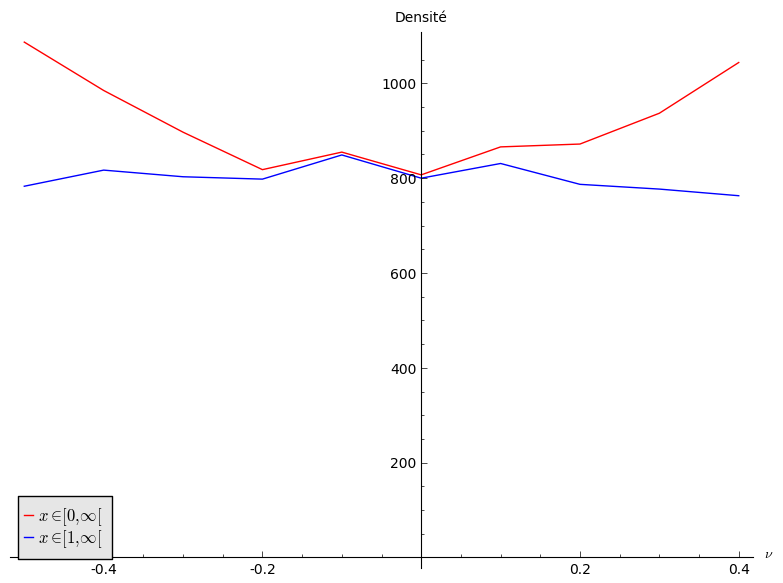Le dessin semble indiquer que la densité non uniforme semble provenir simplement par les points $$(x,\nu)$$ tels que $$x\leq 1$$.

On dessine des histogrammes superposés de la densité de ces points une fois projetés sur l'axe des $$x$$ (on donne des couleurs selon la valeur de $$\nu$$):

sage: histogrammes_x(30000, nbox=5, ymax=1500, xmax=8)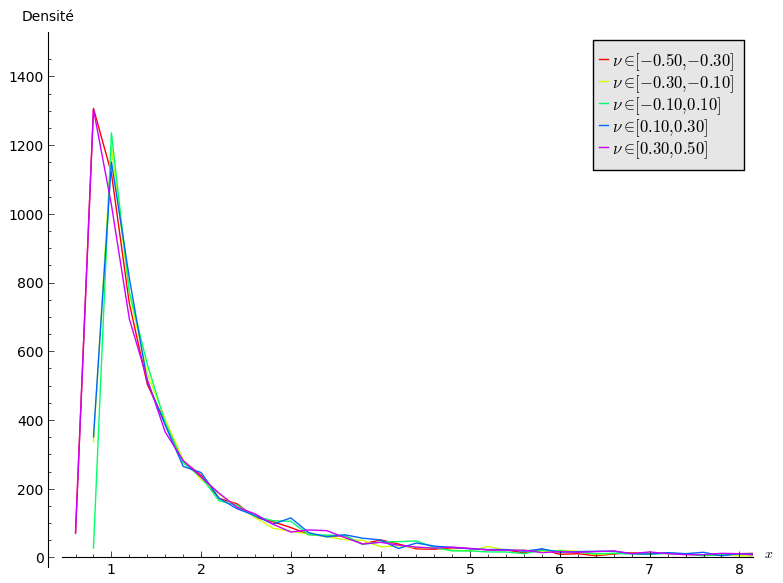Le dessin semble indiquer que la densité ne dépend pas de $$\nu$$ pour $$x\geq 1$$.

]]>
Sébastien Labbé http://www.slabbe.org/blogue <![CDATA[Using sage in the new ipython notebook]]> http://www.slabbe.org/blogue/2013/02/using-sage-in-the-new-ipython-notebook 2013-02-10T11:25:00Z 2013-02-10T11:25:00Z

(NEW: See also the demo I made at the Sage Paris group meeting in March 2014.)

Ticket #12719 (Upgrade to IPython 0.13) was merged into sage-5.7.beta1. This took a lot of energy (see the number of comments in the ticket and especially the number of patches and dependencies). Big thanks to Volker Braun, Mike Hansen, Jason Grout, Jeroen Demeyer who worked on this since almost one year. Note that in December 2012, the IPython project has received a $1.15M grant from the Alfred P. Sloan foundation, that will support IPython development for the next two years. I really like this IPython sage command line interface so it is really good news! The IPython notebook Since version 0.12 (December 21 2011), IPython is released with its own notebook. The differences with the Sage Notebook are explained by Fernando Perez, leader of IPython project, in the blog post The IPython notebook: a historical retrospective he wrote in January 2012. One of the differences is that the IPython Notebook run in its own directory whereas each cell of the Sage Notebook lives in its directory. As William Stein says in the presentation Browser-based notebook interfaces for mathematical software - past, present and future he gave last December at ICERM, there are plenty of projects and directions these days for those interfaces. In May 2012, I tested the same ticket which was to upgrade to IPython 0.12 at that time. Today, I was curious to test it again. First, I installed sage-5.7.beta4: ./sage -version Sage Version 5.7.beta4, Release Date: 2013-02-09 Install tornado: ./sage -sh easy_install-2.7 tornado [update March 6th, 2014] Note that some linux user have to install libssl-dev before tornado: sudo apt-get install libssl-dev Install zeromq and pyzmq: ./sage -i zeromq ./sage -i pyzmq Start the ipython notebook: ./sage -ipython notebook [NotebookApp] Using existing profile dir: u'/Users/slabbe/.sage/ipython-0.12/profile_default' [NotebookApp] Serving notebooks from /Users/slabbe/Applications/sage-5.7.beta4 [NotebookApp] The IPython Notebook is running at: http://127.0.0.1:8888/ [NotebookApp] Use Control-C to stop this server and shut down all kernels.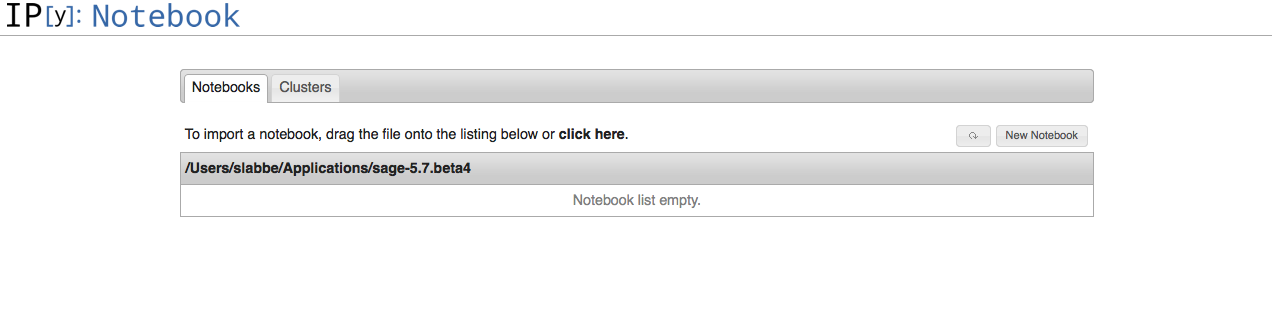Create a new notebook. One may use sage commands by adding the line from sage.all import * in the first cell.The next things I want to look at are: • Test the conversion of files from .py to .pynb. • Test the conversion of files from .rst to .pynb. • Test the qtconsole. • Test the parallel computing capacities of the IPython. ]]> Sébastien Labbé http://www.slabbe.org/blogue <![CDATA[Understanding Python class inheritance and Sage coding convention with fruits]]> http://www.slabbe.org/blogue/2013/01/understanding-python-class-inheritance-and-sage-coding-convention-with-fruits 2013-01-28T11:00:00Z 2013-01-28T11:00:00Z Since Sage Days 20 at Marseille held in January 2010, I have been doing the same example over and over again each time I showed someone else how object oriented coding works in Python: using fruits, strawberry, oranges and bananas.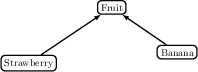Here is my file: fruit.py. I use to build it from scratch by adding one line at a time using attach command to see what has changed starting with Banana class, then Strawberry class then Fruit class which gathers all common methods. This time, I wrote the complete documentation (all tests pass, coverage is 100%) and followed Sage coding convention as far as I know them. Thus, I hope this file can be useful as an example to explain those coding convention to newcomers. One may check that all tests pass using:$ sage -t fruit.py
sage -t  "fruit.py"
[3.7 s]

----------------------------------------------------------------------
All tests passed!
Total time for all tests: 3.8 seconds

One may check that documentation and doctest coverage is 100%:

$sage -coverage fruit.py ---------------------------------------------------------------------- fruit.py SCORE fruit.py: 100% (10 of 10) ---------------------------------------------------------------------- ]]> Sébastien Labbé http://www.slabbe.org/blogue <![CDATA[Python function vs Symbolic function in Sage]]> http://www.slabbe.org/blogue/2013/01/python-function-vs-symbolic-function-in-sage 2013-01-21T08:00:00Z 2013-01-21T08:00:00Z This message is about differences between a Python function and a symbolic function. This is also explained in the Some Common Issues with Functions page in the Sage Tutorial. In Sage, one may define a symbolic function like: sage: f(x) = x^2-1 And draw it using one the following way (both works): sage: plot(f, (x,-10,10)) sage: plot(f(x), (x,-10,10))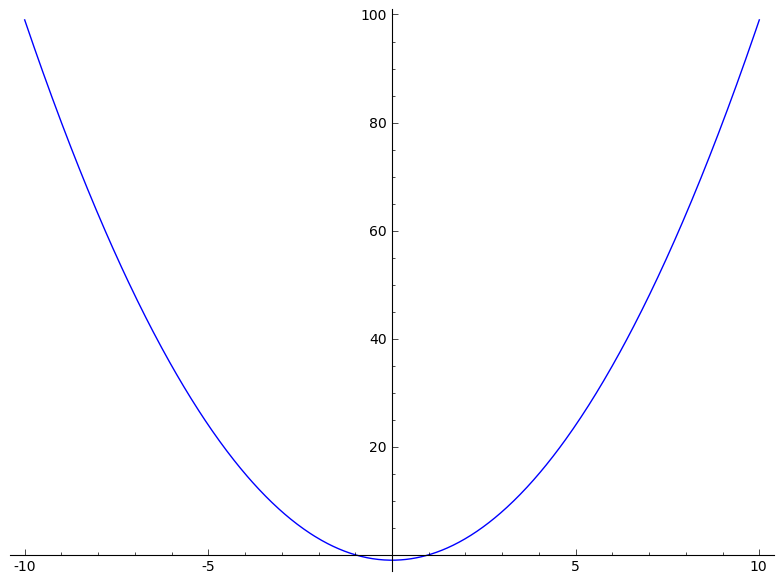Here both f and f(x) are symbolic expressions: sage: type(f) <type 'sage.symbolic.expression.Expression'> sage: type(f(x)) <type 'sage.symbolic.expression.Expression'> although there are different: sage: f x |--> x^2 - 1 sage: f(x) x^2 - 1 Now if f is a Python function defined with a def statement: sage: def f(x): ....: if x>0: ....: return x ....: else: ....: return 0 It is really a Python function: sage: f <function f at 0xb933470> sage: type(f) <type 'function'> As above, one can draw the function f: sage: plot(f, (x,-10,10))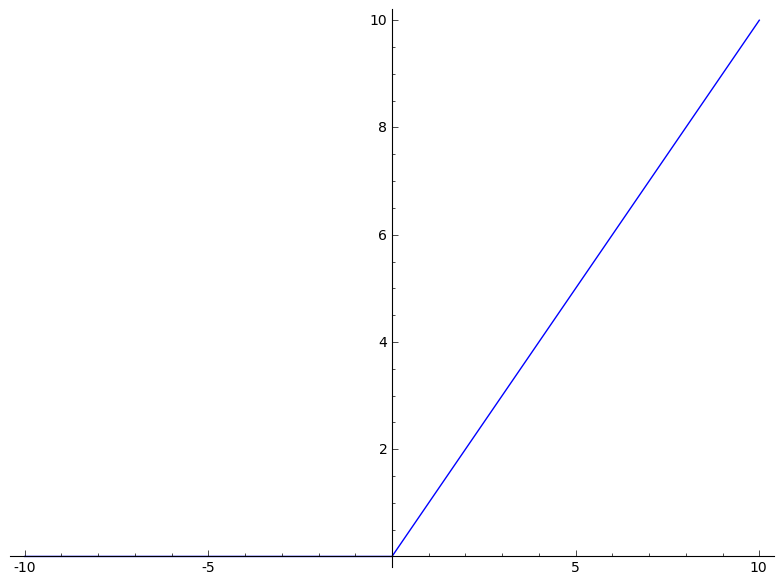But be carefull, drawing f(x) will not work as expected: sage: plot(f(x), (x,-10,10))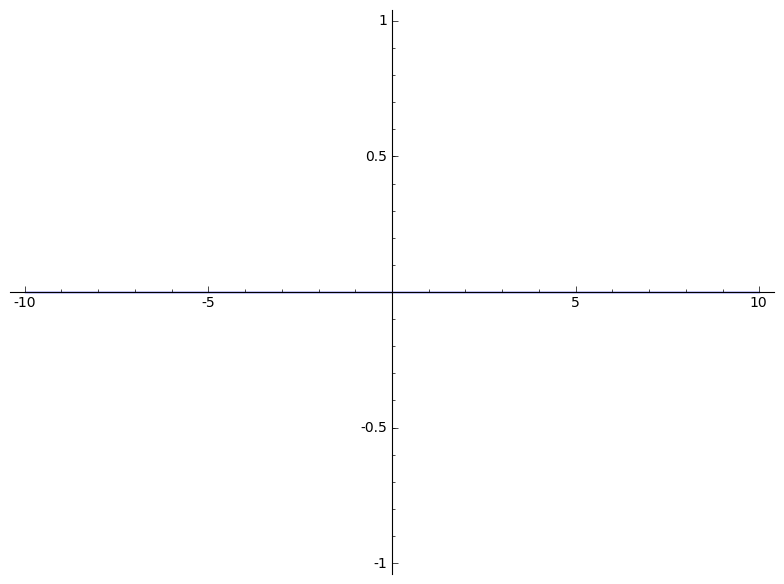Why? Because, the python function f gets evaluated on the variable x and this may either raise an exception depending on the definition of f or return some result which might not be a symbolic expression. Here f(x) gets always evaluated to zero because in the definition of f, bool(x > 0) returns False: sage: x x sage: bool(x > 0) False sage: f(x) 0 Hence the following constant function is drawn: sage: plot(0, (x,-10,10)) which is not what we want. ]]> Sébastien Labbé http://www.slabbe.org/blogue <![CDATA[Percolation and self-avoiding walks]]> http://www.slabbe.org/blogue/2012/12/percolation-and-self-avoiding-walks 2012-12-20T21:12:00Z 2012-12-18T07:12:00Z Today, I am presenting the Chapter 3 of the book Probability on Graphs of Geoffrey Grimmett during a monthly reading seminar at LIAFA. The title of the chapter is Percolation and self-avoiding walks. I did some computations to improve my intuition on the question. My code is in the following file : bond_percolation.sage. This post is about some of my computations. You might want to test them yourself online using the Sage Cell Server. Basic Definitions Let $$\mathbb{L}^d=(\mathbb{Z}^d,\mathbb{E}^d)$$ be the hypercubic lattice. Let $$p\in[0,1]$$. Each edge $$e\in \mathbb{E}^d$$ is designated either open with probability $$p$$, or closed otherwise, different edges receiving independant states. For $$x,y\in \mathbb{Z}^d$$, we write $$x \leftrightarrow y$$ if there exists an open path joining $$x$$ and $$y$$. For $$x\in \mathbb{Z}^d$$, we consider the open cluster $$C_x$$ containing $$x$$ : $C_x = \{y \in \mathbb{Z}^d : x \leftrightarrow y \}.$ The percolation probability $$\Theta(p)$$ is given by $\Theta(p) = P_p(\vert C_0\vert=\infty).$ Finally, the critical probability is defined as $p_c = \sup\{p : \Theta(p) = 0 \}.$ The question is to compute $$p_c$$. Results in the Chapter give lower bound and upper bound for $$p_c$$. Many problems are still open like the one claiming that $$\Theta(p_c) = 0$$ for all $$d\geq 2$$: it is known only for $$d=2$$ and $$d\geq 19$$ according to a remark in the chapter. Some samples when p=0.5 A bond percolation sample inside the box $$\Lambda(m)=[-m,m]^d$$ when $$p=0.5$$ and $$d=2$$: sage: S = BondPercolationSample(p=0.5, d=2) sage: S.plot(m=40, pointsize=10, thickness=1) Graphics object consisting of 7993 graphics primitives sage: _.show()Another time gives something different: sage: S = BondPercolationSample(p=0.5, d=2) sage: S.plot(m=40, pointsize=10, thickness=1) Graphics object consisting of 10176 graphics primitives sage: _.show()Some samples for ranges of values of p From p=0.1 to p=0.9: sage: percolation_graphics_array(srange(0.1,1,0.1), d=2, m=5)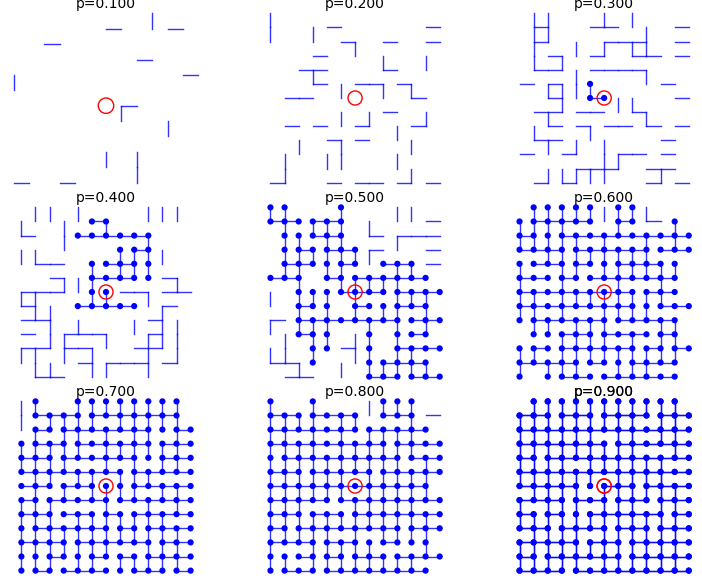From p=0.41 to p=0.49: sage: percolation_graphics_array(srange(0.41,0.50,0.01), d=2, m=5)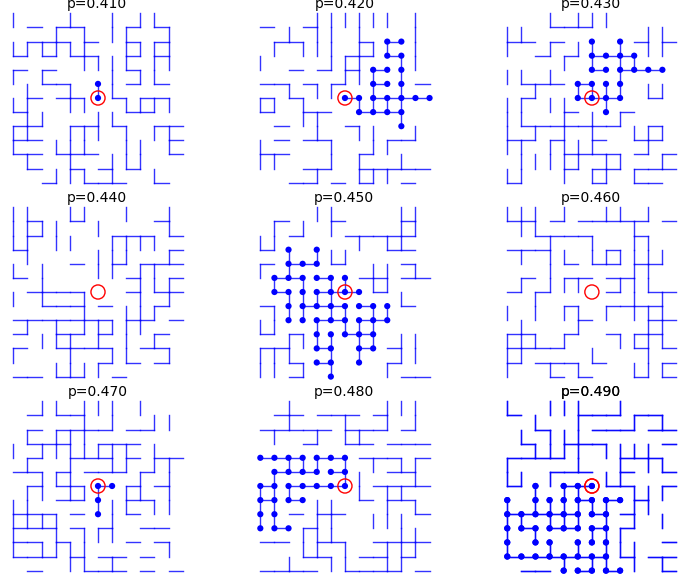From p=0.51 to p=0.59: sage: percolation_graphics_array(srange(0.51,0.60,0.01), d=2, m=5)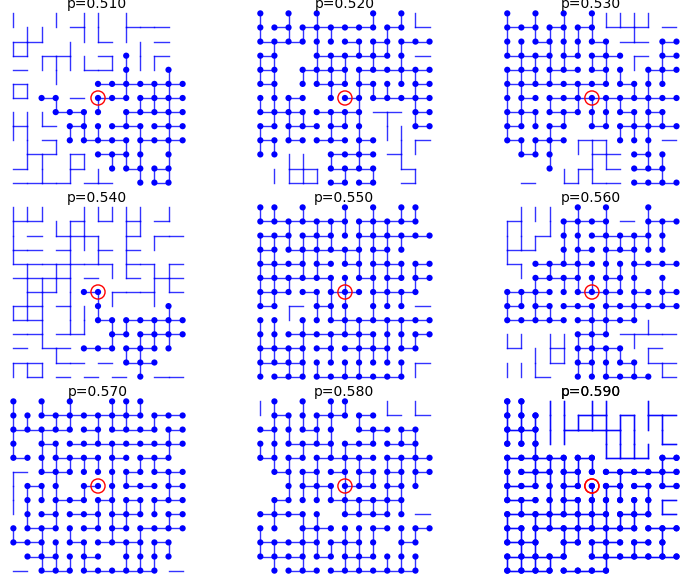Upper bound and lower bound for percolation probability $$\Theta(p)$$ In every case, we have the following upper bound for the percolation probability: $\Theta(p) = \mathbb{P}_p(\vert C_0\vert=\infty) \leq \mathbb{P}_p(\vert C_0\vert > 1) = 1 - \mathbb{P}_p(\vert C_0\vert = 1) = 1 - (1-p)^{2d}.$ In particular, if $$p\neq 1$$, then $$\Theta(p)<1$$. In Sage, define the upper bound: sage: p,n = var('p,n') sage: d = var('d') sage: upper_bound = 1 - (1-p)^(2*d) Also, from Equation (3.8), we have the following lower bound: $\Theta(p) \geq 1 - \sum_{n=4}^{\infty} n (4(1-p))^n.$ In Sage, define the lower bound: sage: p,n = var('p,n') sage: lower_bound = 1 - sum(n*(4*(1-p))^n,n,4,oo) sage: lower_bound.factor() -(3072*p^5 - 14336*p^4 + 26624*p^3 - 24592*p^2 + 11288*p - 2057)/(4*p - 3)^2 This is not defined when $$p=3/4$$, but we are interested in the values in the interval $$]3/4,1]$$. In particular, for which value of $$p$$ is this lower bound strictly larger than zero: sage: root = lower_bound.find_root(0.76, 0.99); root 0.8639366490304586 Let's now draw a graph of the lower and upper bound: sage: U = plot(upper_bound(d=2),(0,1),color='red', thickness=3) sage: L = plot(lower_bound,(0.86,1),color='green', thickness=3) sage: G = U + L sage: G += point((root, 0), color='red', size=20) sage: lower = r"$1-\sum_{n=4}^{\infty} n4^n(1-p)^n$" sage: upper = r"$1 -(1-p)^{4}$" sage: title = r"$1-\sum_{n=4}^{\infty} n4^n(1-p)^n\leq\Theta(p)\leq 1 -(1-p)^{2d}\$"
sage: G += text(title, (.5, 1.05), color='black', fontsize=15)
sage: G += text(upper, (.3, 0.5), color='red', fontsize=20)
sage: G += text(lower, (.7, 0.5), color='green', fontsize=20)
sage: G += text("%.5f"%root,(0.88, .03), color='green', horizontal_alignment='left')
sage: G.show()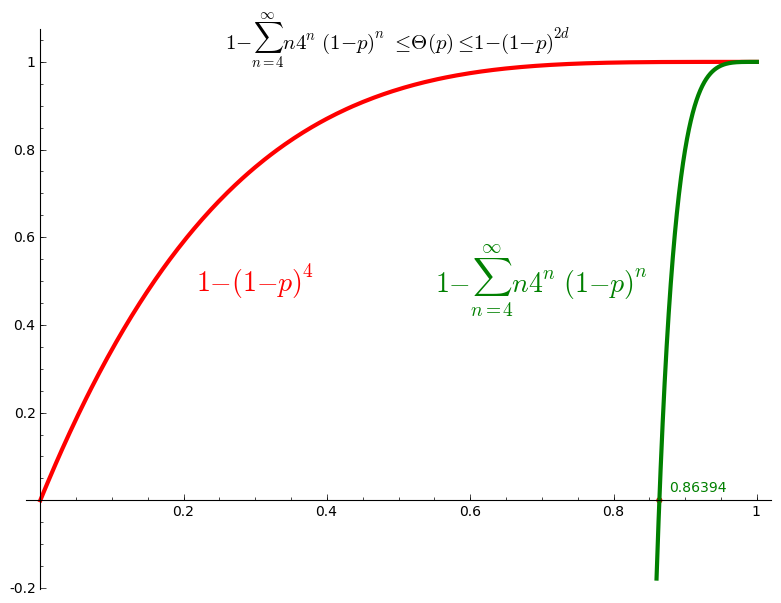Thus we conclude that $$\Theta(p) >0$$ for $$p>0.8639$$ and thus $$p_c \leq 0.8639$$.

Percolation probability - dimension 2

The code allows to define the percolation probability function for a given dimension d. It generates n samples and consider the cluster to be infinite if its cardinality is larger than the given stop value.

Here we use Sage adaptative recursion algorithm for drawing the plot of the percolation probability which finds the particular important intervals to ask for more values of the function. See help section of plot function for details. Because T might be long to compute we start with only 4 points.

When stop=100:

sage: T = PercolationProbability(d=2, n=10, stop=100)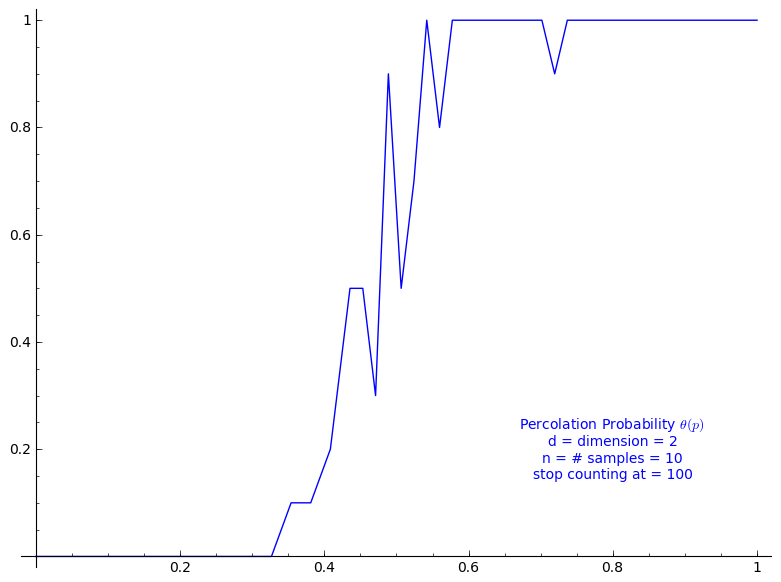When stop=1000:

sage: T = PercolationProbability(d=2, n=10, stop=1000)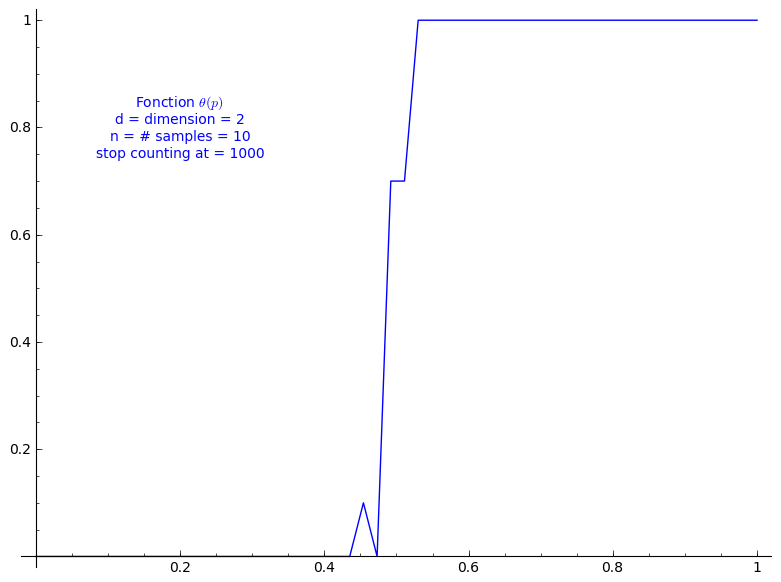When stop=2000:

sage: T = PercolationProbability(d=2, n=10, stop=2000)Percolation probability - dimension 3

When stop=100:

sage: T = PercolationProbability(d=3, n=10, stop=100)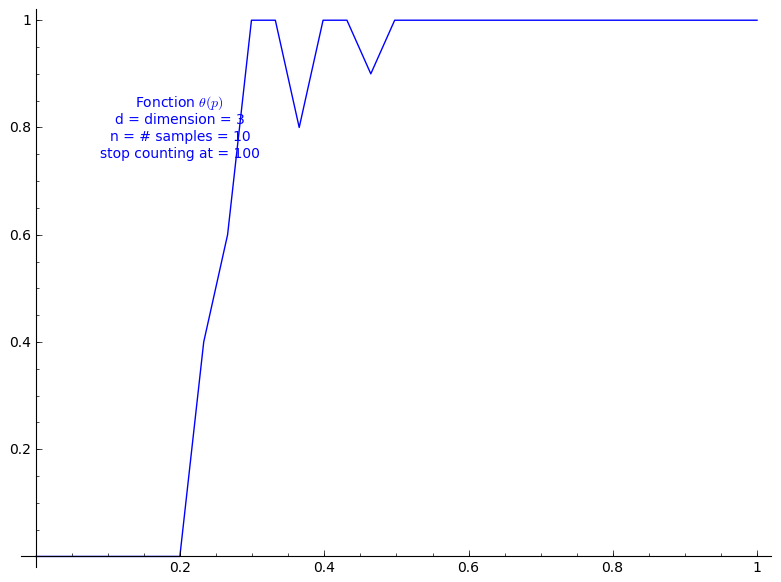When stop=1000:

sage: T = PercolationProbability(d=3, n=10, stop=1000)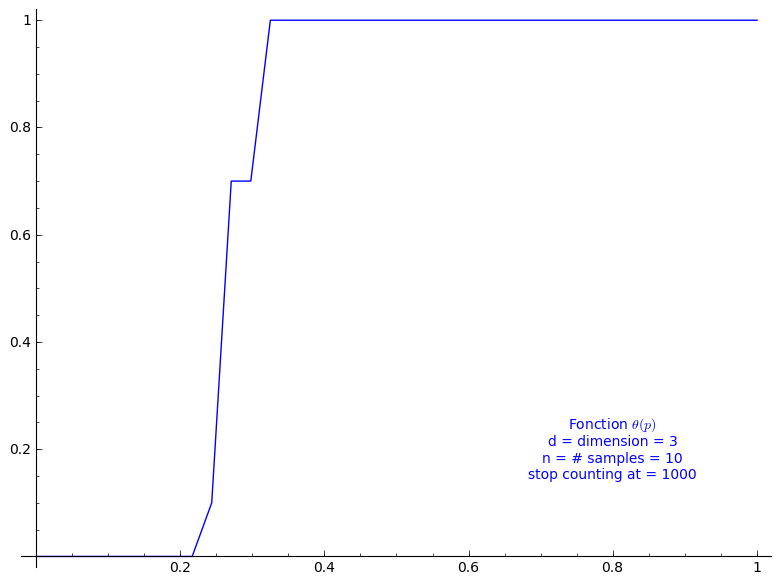Percolation probability - dimension 4

When stop=100:

sage: T = PercolationProbability(d=4, n=10, stop=100)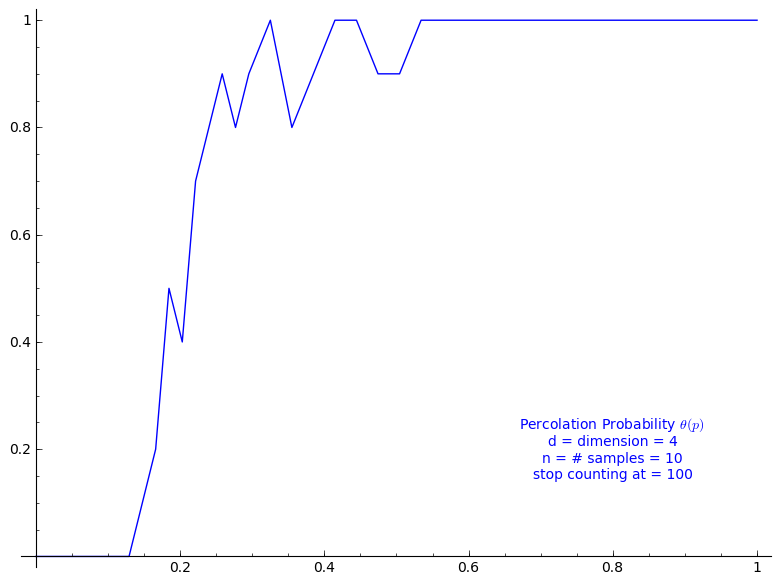Percolation probability - dimension 13

When stop=100:

sage: T = PercolationProbability(d=13, n=10, stop=100)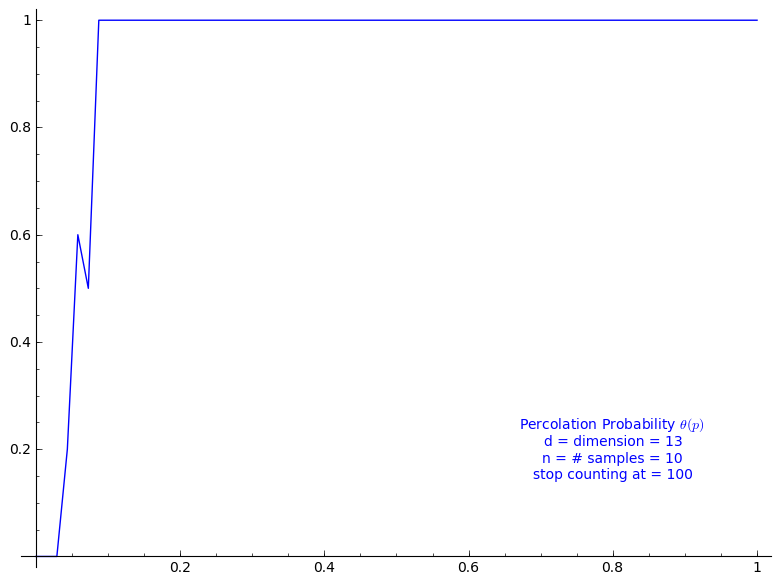Theorem 3.2

Theorem 3.2 states that $$0 < p_c < 1$$, but its proof does much more in fact. Following the computation we just did for Equation (3.8), we get for $$d=2$$ $0.3333 < \frac{1}{2d-1} \leq p_c \leq 0.8639$ and for $$d=3$$: $0.2000 < \frac{1}{2d-1} \leq p_c \leq 0.8639$ This allows to grasp the improvement brought later by Theorem 3.12.

Connective constant

Using the two following sequences of the On-Line Encyclopedia of Integer Sequences, one can evaluate the connective constant $$\kappa(d)$$

• A001411: Number of n-step self-avoiding walks on square lattice.
• A001412: Number of n-step self-avoiding walks on cubic lattice.

By taking the k-th root of of k-th term of A001411, we may give an approximation of $$\kappa(2)$$:

sage: L = [1, 4, 12, 36, 100, 284, 780, 2172, 5916, 16268, 44100, 120292,
324932, 881500, 2374444, 6416596, 17245332, 46466676, 124658732, 335116620,
897697164, 2408806028, 6444560484, 17266613812, 46146397316, 123481354908,
329712786220, 881317491628]
sage: for k in range(1, len(L)): print numerical_approx(L[k]^(1/k))
4.00000000000000
3.46410161513775
3.30192724889463
3.16227766016838
3.09502148400370
3.03400133198980
2.99705187539871
2.96144397263395
2.93714926770637
2.91369345857619
2.89627439045790
2.87949308754677
2.86632078916860
2.85362749495679
2.84328447096562
2.83329615650289
2.82493415671599
2.81684125361654
2.80992368218258
2.80321554383456
2.79738645741910
2.79172363211806
2.78673687369245
2.78188437392354
2.77756387722633
2.77335345579129
2.76956977331575

By taking the k-th root of of k-th term of A001412, we may give an approximation of $$\kappa(3)$$:

sage: L = [1, 6, 30, 150, 726, 3534, 16926, 81390, 387966, 1853886,
8809878, 41934150, 198842742, 943974510, 4468911678, 21175146054,
100121875974, 473730252102, 2237723684094, 10576033219614, 49917327838734,
235710090502158, 1111781983442406, 5245988215191414, 24730180885580790,
116618841700433358, 549493796867100942,2589874864863200574,
12198184788179866902, 57466913094951837030, 270569905525454674614]
sage: for k in range(1, len(L)): print numerical_approx(L[k]^(1/k))
6.00000000000000
5.47722557505166
5.31329284591305
5.19079831727404
5.12452137580198
5.06709510955294
5.02933019629493
4.99573287588832
4.97111339009676
4.94876680377358
4.93129192790635
4.91521453865211
4.90209314463520
4.88990167518413
4.87964724632057
4.87004597517131
4.86178722582108
4.85400655861169
4.84719703702142
4.84074902256992
4.83502763526502
4.82958688248615
4.82470487210973
4.82004549244633
4.81582557693112
4.81178552451599
4.80809774735294
4.80455755518719
4.80130435575213
4.79817388859565

Then, $$\kappa(2)$$ would be something less than 2.769 and $$\kappa(3)$$ would be something less than 4.798.

Theorem 3.12

Thus, we may evaluate the lower bound and upper bound given at Theorem 3.12. For dimension $$d=2$$:

sage: k < 2.76956977331575
k < 2.76956977331575
sage: _ / (2.76956977331575  * k)
0.361066909970928 < (1/k)
sage: 1 - 0.361066909970928
0.638933090029072

The critical probability of bond percolation on $$\mathbb{L}^d$$ with $$d=2$$ satisfies $0.3610 < \frac{1}{\kappa(2)} \leq p_c \leq 1 - \frac{1}{\kappa(2)} < 0.6389.$ If we look at the graph of the percolation probability $$\Theta(p)$$ we did above for when $$d=2$$, it seems that the lower bound is not far from $$p_c$$. The lower bound 0.3610 is a small improvement to the simple one got from Theorem 3.2 (0.3333).

Similarly, for dimension $$d=3$$:

sage: k < 4.79817388859565
k < 4.79817388859565
sage: _ / (4.79817388859565 * k)
0.208412621805310 < (1/k)

The critical probability of bond percolation on $$\mathbb{L}^d$$ with $$d=3$$ satisfies $0.2084 < \frac{1}{\kappa(3)} \leq p_c \leq 1 - \frac{1}{\kappa(2)} < 0.6389.$ Again, if we look at the graph of $$\Theta(p)$$ we did above for when $$d=3$$, it seems that the lower bound 0.2084 is not far from $$p_c$$. In this case, the lower bound 0.2084 is a rather small improvement to the lower bound from Theorem 3.2 (0.2000). It might be caused by a poor approximation of $$\kappa(3)$$ from the above sequences of only 30 terms from the OEIS.

]]>
Sébastien Labbé http://www.slabbe.org/blogue <![CDATA[Some small Sage tricks]]> http://www.slabbe.org/blogue/2012/12/some-small-sage-tricks 2012-12-14T08:00:00Z 2012-12-14T08:00:00Z

Below are some Sage tricks that I gathered from other users of Sage, from sage-devel and other places since one year.

Stop the focus in the Notebook

This Notebook hack of the day was published on sage-devel by William Stein during May 2012 to fix that focus movement in the Notebook:

html('<script>cell_focus=function(x,y){} </script>')

Consult the documentation of a function in the browser

Open the documentation of a particular function in a web browser, from either the command-line or the notebook:

sage: browse_sage_doc(factor)

It works also for methods of an object:

sage: m = matrix(2, range(4))
sage: browse_sage_doc(m.inverse)

I found this command when I consulted the help():

sage: help()

Implicit multiplication

Some behavior in Sage can be made implicit like multiplication and variable definition. This might be good for new users coming from Maple for instance.

Normally, this syntax raises an error:

sage: 34x
------------------------------------------------------------
File "<ipython console>", line 1
34x
^
SyntaxError: invalid syntax

It is possible to make it work:

sage: implicit_multiplication(True)
sage: 34x
34*x

Implicit variable definition

The following works only in the Sage Notebook. It allows to turn on automatic definition of variables:

sage: automatic_names(True)
sage: x + y + z
x + y + z

Turn out automatic show when using plot

Set the default to False for showing plots using any plot commands:

sage: show_default(False)

I prefer False but you may not.

Rerun the patchbot

One can re-run the tests of a particular ticket by adding ?kick to the url of the ticket on the patchbot Sage server. For example, to rerun the tests on the ticket #13461, one can load the following page:

http://patchbot.sagemath.org/ticket/13461/?kick

This trick was shared on sage-devel during August 2012.

Python debugger

The majority of the Best Practices for Scientific Computing are followed by the Sage Development model. But, there is one principle that at least I do not use enough : a debugger. However, a Python debugger exists and a tutorial for using the Python debugger is available on onlamp.com.

I remember that discussion on sage-devel Poll: which debugger do you use? where developers were sharing their debugger tricks. It seems that using print statements is unavoidable.

Computing basic statistics with R

I always knew R is in Sage but never used it even if sometimes I need to compute some statistics of list of numbers. In Sage, one can print statistics of a list of numbers using R:

sage: L = [randint(0,100) for _ in range(20)]
sage: r.summary(L)
Min. 1st Qu.  Median    Mean 3rd Qu.    Max.
14.00   27.75   57.50   53.45   69.00   98.00

Creation of the number field in $$\sqrt{5}$$

Before learning it from this video of William Stein, I did not know that the square brackets could be used to create such number field:

sage: A = ZZ[sqrt(5)]
sage: a,sqrt5 = A.gens()
sage: A
Order in Number Field in sqrt5 with defining polynomial x^2 - 5
sage: sqrt5
sqrt5

Using Sage locally in the notebook from a server

First, log into the server using the following port setup:

ssh -L 8389:localhoat:8389 [SERVER]

Start the notebook with the given port:

sage -notebook port=8389

This ask for a password, if you forget it, you may reset it by first opening Sage, and by starting the notebook with the option reset=True:

sage: notebook(port=8389, reset=True)

Then, by opening the browser at the following adress, I can log in to the notebook from the server:

Going from Mercurial to Git

Sage will soon move from Mercurial to Git. In the past, I tried to understand the difference between Mercurial and Git. I was never able to find a simple text or blog post on the web explaining in a simple way the differences. One diffence would be about branching. But I was not using branches with Mercurial... As I see it, differences between Mercurial and Git are hard to explain or at least hard to understand.

Anyway, I once found this tutorial on the Git version control system: Understanding Git Conceptually where the approach is "conceptual" and maybe easier for the mathematician. For instance, in Section 1 it is written that repositories can be "visualized as a directed acyclic graph of commit objects". I still haven't go through this tutorial but I consider to start with this one.

Inheritance tree

One may draw the inheritance tree of a class with the following command:

sage: class_graph(Integer).plot()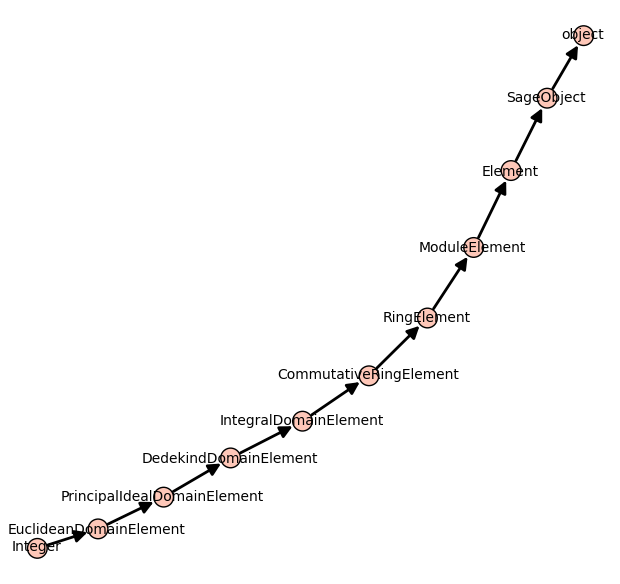]]>
Sébastien Labbé http://www.slabbe.org/blogue <![CDATA[Using Sage + graphviz + dot2tex + tikz + tikz2pdf to draw a graph]]> http://www.slabbe.org/blogue/2012/11/using-sage-graphviz-dot2tex-tikz-tikz2pdf-to-draw-a-graph 2012-11-16T08:00:00Z 2012-11-13T08:00:00Z

Let's first construct a graph that we will use in our examples below. We first construct a finite group generated by 2 by 2 matrices on the field $$GF(3)$$. The group contains 24 elements. We then construct its Cayley graph:

sage: F = GF(3)
sage: gens = [matrix(F,2,[1,0, 1,1]), matrix(F,2, [1,1, 0,1])]
sage: group = MatrixGroup(gens); group
Matrix group over Finite Field of size 3 with 2 generators:
[[[1, 0], [1, 1]], [[1, 1], [0, 1]]]
sage: group.cardinality()
24
sage: G = group.cayley_graph()

Default graph plot

The default graph plot in Sage is:

sage: G.show(color_by_label=True)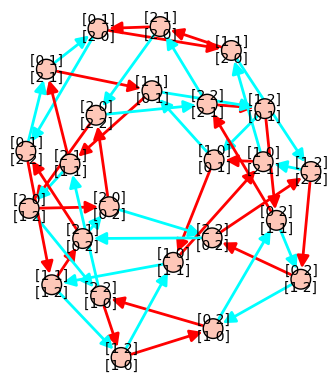Using view is actually broken when vertices are matrices because default format (format='tkz_graph') does not support it:

sage: view(G)
An error occurred.
...
LaTex error

Installing dot2tex + graphviz

One may get another kind of tikz output using the dot2tex.spkg together with graphviz. To know what is the latest available version of dot2tex use the command optional_packages():

sage: [x for x in flatten(optional_packages()) if 'dot2tex' in x]
['dot2tex-2.8.7-2']

The command to install the most recent version of dot2tex.spkg do from the command line (where you replace the version numbers by the above output):

sage -i dot2tex-2.8.7-2

As the documentation of G.layout_graphviz() says, install graphviz >= 2.14 so that the programs dot, neato, ... are in your path. The graphviz suite can be download from the graphviz website.

Basic Usage

This should allow the following to work:

sage: G.set_latex_options(format='dot2tex', prog='neato')
sage: G.set_latex_options(color_by_label=True)
sage: view(G)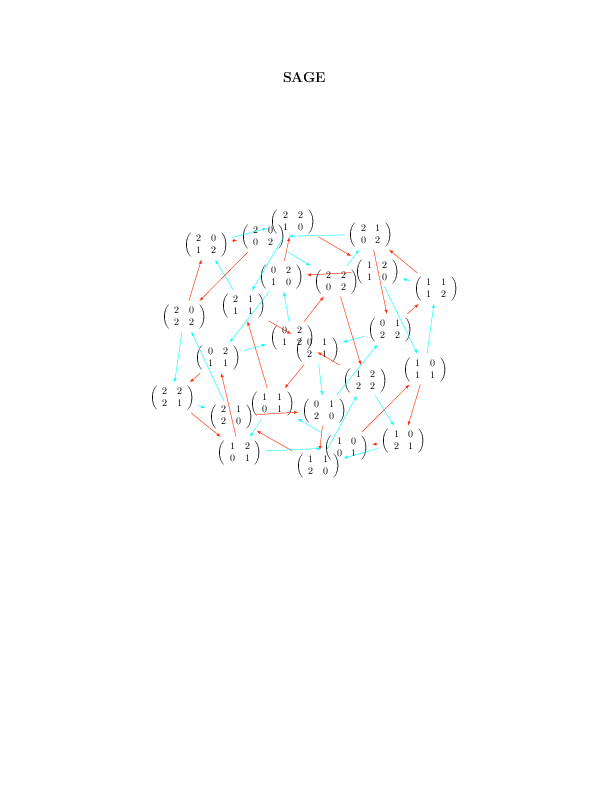Limits of the basic usage

With the above usage, you will find that the command view(G) command is very slow and that sometimes it just doesn't work and gives a Latex error like this:

sage: G.set_latex_options(format='dot2tex', prog='dot')
sage: view(G)
An error occurred.
...
LaTex error

This is because the default compilation is just unappropriate for our usage (I still wonder for which usage it can be appropriate). In fact, the default compilation is first trying the conversion tex to dvi to png using latex and dvipng. If the dvipng part does not work for whatever reason (which is our case), it will then try the conversion dvi to ps to pdf using dvips and ps2pdf. This worked above for prog='neato' but not for prog='dot' because dvipng does not seem to like when latex produces Overfull \hbox and Overfull \vbox.

The Best Usage

The compilation strategy can be changed by using the engine option and by setting it to 'pdflatex'. Also the Overfull problem can be solved using the option tightpage=True:

sage: G.set_latex_options(format='dot2tex', prog='dot')
sage: G.set_latex_options(color_by_label=True)
sage: view(G, engine='pdflatex', tightpage=True)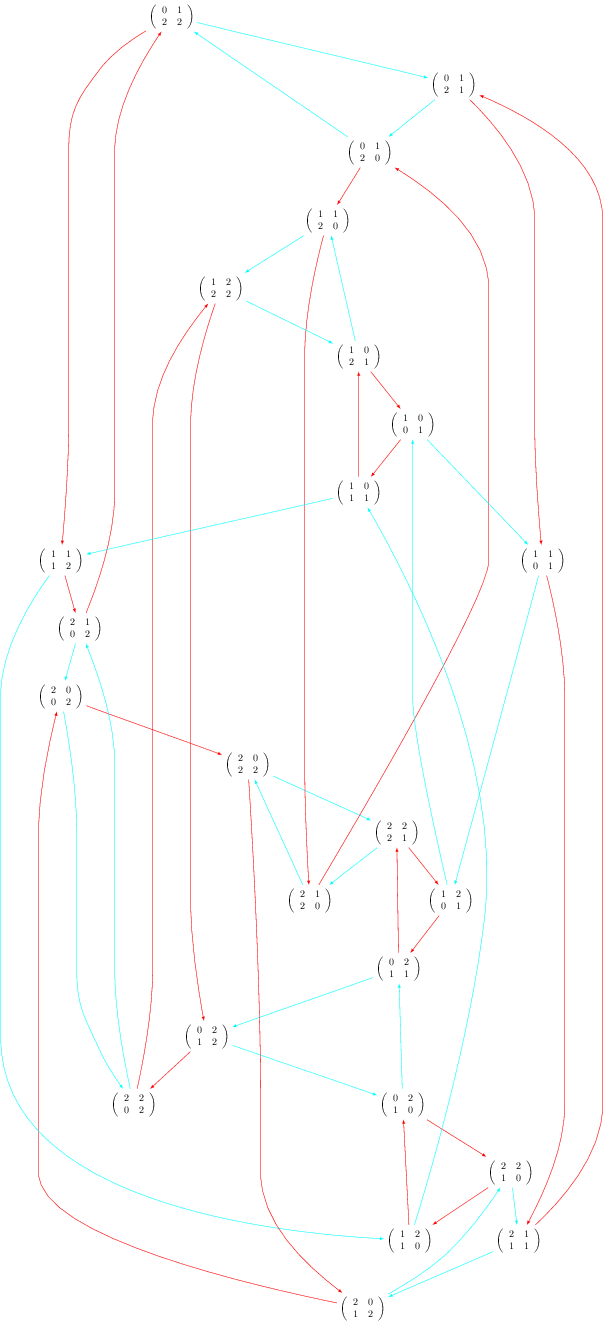More options

The variable prog is for the program used for the layout. It must be a string corresponding to one of the software of the graphviz suite. Accepted values for prog are:

• 'dot' (the default)
• 'neato'
• 'twopi'
• 'circo'
• 'fdp'

When using format='dot2tex', other available options are:

sage: G.set_latex_options(color_by_label=True)
sage: G.set_latex_options(edge_labels=True)
sage: G.set_latex_options(edge_colors=I_dont_know_what)

Consult the help for more details:

sage: opts = G.latex_options()
sage: opts.set_option?

However, these other options do not seem to work perfectly. I don't know what format to give to edges_colors and edge_labels=True seems broken. I posted a workaround on the sagetrac to fix it.

Using the tikz2pdf script instead of the command view

Alternatively, when I get problems with the view command, I use my script tikz2pdf instead:

sage: G.set_latex_options(format='dot2tex', prog='dot')
sage: G.set_latex_options(color_by_label=True)
sage: G.latex_options()
LaTeX options for Digraph on 24 vertices: {'prog': 'dot', 'color_by_label': True, 'format': 'dot2tex'}
sage: s = G.latex_options().dot2tex_picture()
sage: f = open('graph_dot.tikz', 'w')
sage: f.write(s)
sage: f.close()
sage: !tikz2pdf graph_dot.tikz
Using template ...
tikz2pdf: calling pdflatex...
tikz2pdf: Output written to 'graph_dot.pdf'.
]]>